# US20120269190A1 - VLSI Layouts of Fully Connected Generalized and Pyramid Networks with Locality Exploitation - Google Patents

VLSI Layouts of Fully Connected Generalized and Pyramid Networks with Locality Exploitation Download PDF

## Info

Publication number
US20120269190A1
US20120269190A1 US13/502,207 US201013502207A US2012269190A1 US 20120269190 A1 US20120269190 A1 US 20120269190A1 US 201013502207 A US201013502207 A US 201013502207A US 2012269190 A1 US2012269190 A1 US 2012269190A1
Authority
US
United States
Prior art keywords
block
switch
stage
switches
Prior art date
Legal status (The legal status is an assumption and is not a legal conclusion. Google has not performed a legal analysis and makes no representation as to the accuracy of the status listed.)
Granted
Application number
US13/502,207
Other versions
US8898611B2 (en
Inventor
Venkat Konda
Current Assignee (The listed assignees may be inaccurate. Google has not performed a legal analysis and makes no representation or warranty as to the accuracy of the list.)
Konda Tech Inc
Original Assignee
Konda Tech Inc
Priority date (The priority date is an assumption and is not a legal conclusion. Google has not performed a legal analysis and makes no representation as to the accuracy of the date listed.)
Filing date
Publication date
Family has litigation
Application filed by Konda Tech Inc filed Critical Konda Tech Inc
Priority to US13/502,207 priority Critical patent/US8898611B2/en
Publication of US20120269190A1 publication Critical patent/US20120269190A1/en
Assigned to Konda Technologies Inc. reassignment Konda Technologies Inc. ASSIGNMENT OF ASSIGNORS INTEREST (SEE DOCUMENT FOR DETAILS). Assignors: KONDA, VENKAT
Application granted granted Critical
Publication of US8898611B2 publication Critical patent/US8898611B2/en
Priority to US16/671,177 priority patent/US10977413B1/en
Priority to US17/167,082 priority patent/US11451490B1/en
Priority to US17/938,928 priority patent/US11811683B1/en
Active legal-status Critical Current

## Images

•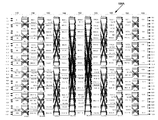•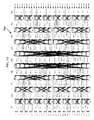•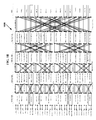•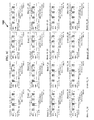••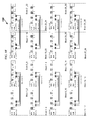•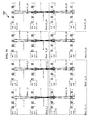•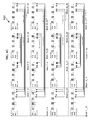•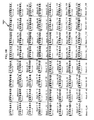•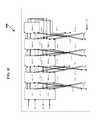••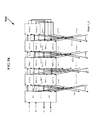•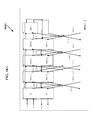•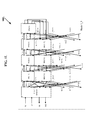••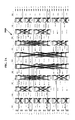•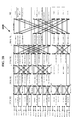•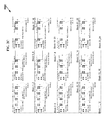•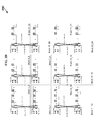•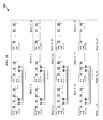•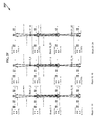•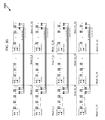••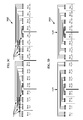••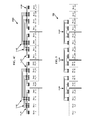•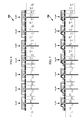••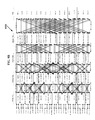••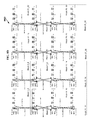•••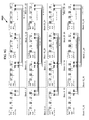•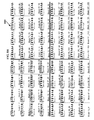•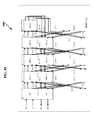•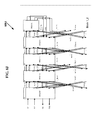••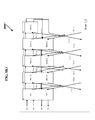•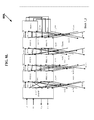•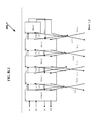•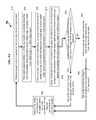•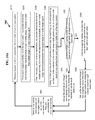•## Classifications

• HELECTRICITY
• H04ELECTRIC COMMUNICATION TECHNIQUE
• H04LTRANSMISSION OF DIGITAL INFORMATION, e.g. TELEGRAPHIC COMMUNICATION
• H04L49/00Packet switching elements
• H04L49/15Interconnection of switching modules
• H04L49/1515Non-blocking multistage, e.g. Clos
• GPHYSICS
• G06COMPUTING; CALCULATING OR COUNTING
• G06FELECTRIC DIGITAL DATA PROCESSING
• G06F30/18Network design, e.g. design based on topological or interconnect aspects of utility systems, piping, heating ventilation air conditioning [HVAC] or cabling
• GPHYSICS
• G06COMPUTING; CALCULATING OR COUNTING
• G06FELECTRIC DIGITAL DATA PROCESSING
• G06F30/30Circuit design
• G06F30/34Circuit design for reconfigurable circuits, e.g. field programmable gate arrays [FPGA] or programmable logic devices [PLD]
• GPHYSICS
• G06COMPUTING; CALCULATING OR COUNTING
• G06FELECTRIC DIGITAL DATA PROCESSING
• G06F30/30Circuit design
• G06F30/34Circuit design for reconfigurable circuits, e.g. field programmable gate arrays [FPGA] or programmable logic devices [PLD]
• G06F30/347Physical level, e.g. placement or routing

## Abstract

The VLSI layouts with spacial locality exploitation presented are applicable to generalized multi-stage and pyramid networks, generalized folded multi-stage and pyramid networks, generalized butterfly fat tree and pyramid networks, generalized multi-link multi-stage and pyramid networks, generalized folded multi-link multi-stage and pyramid networks, generalized multi-link butterfly fat tree and pyramid networks, generalized hypercube networks, and generalized cube connected cycles networks for speedup of s≧1. The embodiments of VLSI layouts are useful in wide target applications such as FPGAs, CPLDs, pSoCs, ASIC placement and route tools, networking applications, parallel & distributed computing, and reconfigurable computing.

## Description

CROSS REFERENCE TO RELATED APPLICATIONS
• This application is Continuation In Part PCT Application to and incorporates by reference in its entirety the U.S. Provisional Patent Application Ser. No. 61/252,603 entitled “VLSI LAYOUTS OF FULLY CONNECTED NETWORKS WITH LOCALITY EXPLOITATION” by Venkat Konda assigned to the same assignee as the current application, filed Oct. 16, 2009.
• This application is Continuation In Part PCT Application to and incorporates by reference in its entirety the U.S. Provisional Patent Application Ser. No. 61/252,609 entitled “VLSI LAYOUTS OF FULLY CONNECTED GENERALIZED AND PYRAMID NETWORKS” by Venkat Konda assigned to the same assignee as the current application, filed Oct. 16, 2009.
• This application is related to and incorporates by reference in its entirety the U.S. application Ser. No. 12/530,207 entitled “FULLY CONNECTED GENERALIZED MULTI-STAGE NETWORKS” by Venkat Konda assigned to the same assignee as the current application, filed Sep. 6, 2009, the U.S. Provisional Patent Application Ser. No. 60/905,526 entitled “LARGE SCALE CROSSPOINT REDUCTION WITH NONBLOCKING UNICAST & MULTICAST IN ARBITRARILY LARGE MULTI-STAGE NETWORKS” by Venkat Konda assigned to the same assignee as the current application, filed Mar. 6, 2007, and the U.S. Provisional Patent Application Ser. No. 60/940,383 entitled “FULLY CONNECTED GENERALIZED MULTI-STAGE NETWORKS” by Venkat Konda assigned to the same assignee as the current application, filed May 25, 2007.
• This application is related to and incorporates by reference in its entirety the U.S. application Ser. No. 12/601,273 entitled “FULLY CONNECTED GENERALIZED BUTTERFLY FAT TREE NETWORKS” by Venkat Konda assigned to the same assignee as the current application, filed Nov. 22, 2009, the U.S. Provisional Patent Application Ser. No. 60/940,387 entitled “FULLY CONNECTED GENERALIZED BUTTERFLY FAT TREE NETWORKS” by Venkat Konda assigned to the same assignee as the current application, filed May 25, 2007, and the U.S. Provisional Patent Application Ser. No. 60/940,390 entitled “FULLY CONNECTED GENERALIZED MULTI-LINK BUTTERFLY FAT TREE NETWORKS” by Venkat Konda assigned to the same assignee as the current application, filed May 25, 2007
• This application is related to and incorporates by reference in its entirety the U.S. application Ser. No. 12/601,274 entitled “FULLY CONNECTED GENERALIZED MULTI-LINK MULTI-STAGE NETWORKS” by Venkat Konda assigned to the same assignee as the current application, filed Nov. 22, 2009, the U.S. Provisional Patent Application Ser. No. 60/940,389 entitled “FULLY CONNECTED GENERALIZED REARRANGEABLY NONBLOCKING MULTI-LINK MULTI-STAGE NETWORKS” by Venkat Konda assigned to the same assignee as the current application, filed May 25, 2007, the U.S. Provisional Patent Application Ser. No. 60/940,391 entitled “FULLY CONNECTED GENERALIZED FOLDED MULTI-STAGE NETWORKS” by Venkat Konda assigned to the same assignee as the current application, filed May 25, 2007 and the U.S. Provisional Patent Application Ser. No. 60/940,392 entitled “FULLY CONNECTED GENERALIZED STRICTLY NONBLOCKING MULTI-LINK MULTI-STAGE NETWORKS” by Venkat Konda assigned to the same assignee as the current application, filed May 25, 2007.
• This application is related to and incorporates by reference in its entirety the U.S. application Ser. No. 12/601,275 entitled “VLSI LAYOUTS OF FULLY CONNECTED GENERALIZED NETWORKS” by Venkat Konda assigned to the same assignee as the current application, filed Nov. 22, 2009, and the U.S. Provisional Patent Application Ser. No. 60/940,394 entitled “VLSI LAYOUTS OF FULLY CONNECTED GENERALIZED NETWORKS” by Venkat Konda assigned to the same assignee as the current application, filed May 25, 2007.
• BACKGROUND OF INVENTION
• Multi-stage interconnection networks such as Benes networks and butterfly fat tree networks are widely useful in telecommunications, parallel and distributed computing. However VLSI layouts, known in the prior art, of these interconnection networks in an integrated circuit are inefficient and complicated.
• Other multi-stage interconnection networks including butterfly fat tree networks, Banyan networks, Batcher-Banyan networks, Baseline networks, Delta networks, Omega networks and Flip networks have been widely studied particularly for self routing packet switching applications. Also Benes Networks with radix of two have been widely studied and it is known that Benes Networks of radix two are shown to be built with back to back baseline networks which are rearrangeably nonblocking for unicast connections.
• The most commonly used VLSI layout in an integrated circuit is based on a two-dimensional grid model comprising only horizontal and vertical tracks. An intuitive interconnection network that utilizes two-dimensional grid model is 2D Mesh Network and its variations such as segmented mesh networks. Hence routing networks used in VLSI layouts are typically 2D mesh networks and its variations. However Mesh Networks require large scale cross points typically with a growth rate of O(N2) where N is the number of computing elements, ports, or logic elements depending on the application.
• Multi-stage interconnection network with a growth rate of O(N×log N) requires significantly small number of cross points. U.S. Pat. No. 6,185,220 entitled “Grid Layouts of Switching and Sorting Networks” granted to Muthukrishnan et al. describes a VLSI layout using existing VLSI grid model for Benes and Butterfly networks. U.S. Pat. No. 6,940,308 entitled “Interconnection Network for a Field Programmable Gate Array” granted to Wong describes a VLSI layout where switches belonging to lower stage of Benes Network are layed out close to the logic cells and switches belonging to higher stages are layed out towards the center of the layout.
• Due to the inefficient and in some cases impractical VLSI layout of Benes and butterfly fat tree networks on a semiconductor chip, today mesh networks and segmented mesh networks are widely used in the practical applications such as field programmable gate arrays (FPGAs), programmable logic devices (PLDs), and parallel computing interconnects. The prior art VLSI layouts of Benes and butterfly fat tree networks and VLSI layouts of mesh networks and segmented mesh networks require large area to implement the switches on the chip, large number of wires, longer wires, with increased power consumption, increased latency of the signals which effect the maximum clock speed of operation. Some networks may not even be implemented practically on a chip due to the lack of efficient layouts.
• SUMMARY OF INVENTION
• When large scale sub-integrated circuit blocks with inlet and outlet links are layed out in an integrated circuit device in a two-dimensional grid arrangement, (for example in an FPGA where the sub-integrated circuit blocks are Lookup Tables) the most intuitive routing network is a network that uses horizontal and vertical links only (the most often used such a network is one of the variations of a 2D Mesh network). A direct embedding of a generalized multi-stage network on to a 2D Mesh network is neither simple nor efficient.
• The VLSI layouts with spacial locality exploitation presented are applicable to generalized multi-stage and pyramid networks V(N1, N2, d, s) & VP(N1, N2, d, s), generalized folded multi-stage and pyramid networks Vfold(N1, N2, d, s) & Vfold-p(N1, N2, d, s), generalized butterfly fat tree and butterfly fat pyramid networks Vbft(N1, N2, d, s) & Vbfp(N1, N2, d, s), generalized multi-link multi-stage and pyramid networks Vmlink(N1, N2, d, s) & Vmlink-p(N1, N2, d, s), generalized folded multi-link multi-stage and pyramid networks Vfold-mlink(N1, N2, d, s) & Vfold-mlink-p(N1, N2, d, s), generalized multi-link butterfly fat tree and butterfly fat pyramid networks Vmlink-bft(N1, N2, d, s) & Vmlink-bfp(N1, N2, d, s), generalized hypercube networks Vhcube(N1, N2, d, s), and generalized cube connected cycles networks VCCC(N1, N2, d, s) for s=1,2,3 or any number in general. The embodiments of VLSI layouts are useful in wide target applications such as FPGAs, CPLDs, pSoCs, ASIC placement and route tools, networking applications, parallel & distributed computing, and reconfigurable computing.
• BRIEF DESCRIPTION OF DRAWINGS
• FIG. 1A is a diagram 100A of an exemplary symmetrical multi-link multi-stage network Vfold-mlink(N, d, s) having a variation of inverse Benes connection topology of nine stages with N=32, d=2 and s=2, strictly nonblocking network for unicast connections and rearrangeably nonblocking network for arbitrary fan-out multicast connections, in accordance with the invention.
• FIG. 1B is a diagram 100B of the equivalent symmetrical folded multi-link multi-stage network Vfold-mlink(N, d, s) of the network 100A shown in FIG. 1A, having a variation of inverse Benes connection topology of five stages with N=32, d=2 and s=2, strictly nonblocking network for unicast connections and rearrangeably nonblocking network for arbitrary fan-out multicast connections, in accordance with the invention.
• FIG. 1C is a diagram 100C layout of the network Vfold-mlink(N, d, s) shown in FIG. 1B, in one embodiment, illustrating the connection links belonging with in each block only.
• FIG. 1D is a diagram 100D layout of the network Vfold-mlink(N, d, s) shown in FIG. 1B, in one embodiment, illustrating the connection links ML(c1,i) for i=[1, 64] and ML(8,i) for i=[1, 64].
• FIG. 1E is a diagram 100E layout of the network Vfold-mlink(N, d, s) shown in FIG. 1B, in one embodiment, illustrating the connection links ML(2,i) for i=[1, 64] and ML(7,i) for i=[1, 64].
• FIG. 1F is a diagram 100F layout of the network Vfold-mlink(N, d, s) shown in FIG. 1B, in one embodiment, illustrating the connection links ML(3,i) for i=[1, 64] and ML(6,i) for i=[1, 64].
• FIG. 1G is a diagram 100G layout of the network Vfold-mlink(N, d, s) shown in FIG. 1B, in one embodiment, illustrating the connection links ML(4,i) for i=[1, 64] and ML(5,i) for i=[1, 64].
• FIG. 1H is a diagram 100H layout of a network Vfold-mlink(N, d, s) where N=128, d=2, and s=2, in one embodiment, illustrating the connection links belonging with in each block only.
• FIG. 1I is a diagram 100I detailed connections of BLOCK 1_2 in the network layout 100C in one embodiment, illustrating the connection links going in and coming out when the layout 100C is implementing Vmliink(N, d, s) or Vfold-mlink(N, d, s).
• FIG. 1J is a diagram 100J detailed connections of BLOCK 1_2 in the network layout 100C in one embodiment, illustrating the connection links going in and coming out when the layout 100C is implementing Vmlink-bft(N, d, s).
• FIG. 1K is a diagram 100K detailed connections of BLOCK 1_2 in the network layout 100C in one embodiment, illustrating the connection links going in and coming out when the layout 100C is implementing V(N, d, s) or Vfold(N, d, s).
• FIG. 1K1 is a diagram 100M1 detailed connections of BLOCK 1_2 in the network layout 100C in one embodiment, illustrating the connection links going in and coming out when the layout 100C is implementing V(N, d, s) or Vfold(N, d, s) for s=1.
• FIG. 1L is a diagram 100L detailed connections of BLOCK 1_2 in the network layout 100C in one embodiment, illustrating the connection links going in and coming out when the layout 100C is implementing Vbft(N, d, s).
• FIG. 1L1 is a diagram 100L1 detailed connections of BLOCK 1_2 in the network layout 100C in one embodiment, illustrating the connection links going in and coming out when the layout 100C is implementing Vbft(N, d, s) for s=1.
• FIG. 2A is a diagram 200A of an exemplary symmetrical multi-link multi-stage network Vfold-mlink(N, d, s) having inverse Benes connection topology of nine stages with N=24, d=2 and s=2, strictly nonblocking network for unicast connections and rearrangeably nonblocking network for arbitrary fan-out multicast connections, in accordance with the invention.
• FIG. 2B is a diagram 200B of the equivalent symmetrical folded multi-link multi-stage network Vfold-mlink(N, d, s) of the network 200A shown in FIG. 2A, having inverse Benes connection topology of five stages with N=24, d=2 and s=2, strictly nonblocking network for unicast connections and rearrangeably nonblocking network for arbitrary fan-out multicast connections, in accordance with the invention.
• FIG. 2C is a diagram 200C layout of the network Vfold-mlink(N, d, s) shown in FIG. 2B, in one embodiment, illustrating the connection links belonging with in each block only.
• FIG. 2D is a diagram 200D layout of the network Vfold-mlink(N, d, s) shown in FIG. 2B, in one embodiment, illustrating the connection links ML(1,i) for i=[1, 48] and ML(8,i) for i=[1, 48].
• FIG. 2E is a diagram 200E layout of the network Vfold-mlink(N, d, s) shown in FIG. 2B, in one embodiment, illustrating the connection links ML(2,i) for i=[1, 32] and ML(7,i) for i=[1,32].
• FIG. 2F is a diagram 200F layout of the network Vfold-mlink(N, d, s) shown in FIG. 2B, in one embodiment, illustrating the connection links ML(3,i) for i=[1, 64] and ML(6,i) for i=[1, 64].
• FIG. 2G is a diagram 200G layout of the network Vfold-mlink(N, d, s) shown in FIG. 2B, in one embodiment, illustrating the connection links ML(4,i) for i=[1, 64] and ML(5,i) for i=[1, 64].
• FIG. 3A is a diagram 300A layout of the topmost row of the network Vfold-mlink(N, d, s) with N=512, d=2 and s=2, in one embodiment, illustrating the provisioning of 2's BW.
• FIG. 3B is a diagram 300B layout of the topmost row of the network Vfold-mlink(N, d, s) with N=512, d=2 and s=2, in one embodiment, illustrating the provisioning of 4's BW.
• FIG. 3C is a diagram 300C layout of the topmost row of the network Vfold-mlink(N, d, s) with N=512, d=2 and s=2, in one embodiment, illustrating the provisioning of 8's BW with nearest neighbor connectivity first.
• FIG. 3D is a diagram 300D layout of the topmost row of the network Vfold-mlink(N, d, s) with N=512, d=2 and s=2, in one embodiment, illustrating the provisioning of 8's BW with nearest neighbor connectivity recursively.
• FIG. 4A is a diagram 400A layout of the topmost row of the network Vfold-mlink(N, d, s) with N=512, d=2 and s=2, in one embodiment, illustrating the provisioning of 2's BW in first stage.
• FIG. 4B is a diagram 400B layout of the topmost row of the network Vfold-mlink(N, d, s) with N=512, d=2 and s=2, in one embodiment, illustrating the remaining nearest neighbor connectivity in the second stage by provisioning 4's BW, 8's BW etc.
• FIG. 4C is a diagram 400C layout of the topmost row of the network Vfold-mlink(N, d, s) with N=512, d=2 and s=2, in one embodiment, illustrating the third stage, by provisioning 4's and 8's BW.
• FIG. 5 is a diagram 500 layout of the topmost row of the network Vfold-mlink(N, d, s) with N=512, d=2 and s=2, in one embodiment, illustrating the provisioning of 8's BW and 16's BW in Partial & Tapered Connectivity (Bandwidth) in a stage.
• FIG. 6 is a diagram 600 layout of the topmost row of the network Vfold-mlink(N, d, s) with N=2048, d=2 and s=2, in one embodiment, illustrating the provisioning of 8's BW, 16's BW and 32's BW in Partial & Tapered Connectivity (Bandwidth) in a stage.
• FIG. 7 is a diagram 700 layout of the topmost row of the network Vfold-mlink(N, d, s) with N=2048, d=2 and s=2, in one embodiment, illustrating the provisioning of 8's BW, 16's BW and 32's BW in Partial & Tapered Connectivity (Bandwidth) in a stage with equal length wires.
• FIG. 8A is a diagram 800A of an exemplary symmetrical multi-link multi-stage pyramid network Vmlink-p(N, d, s) having inverse Benes connection topology of nine stages with N=32, d=2 and s=2, strictly nonblocking network for unicast connections and rearrangeably nonblocking network for arbitrary fan-out multicast connections, in accordance with the invention.
• FIG. 8B is a diagram 800B of the equivalent symmetrical folded multi-link multi-stage pyramid network Vfold-mlink-p(N, d, s) of the network 800A shown in FIG. 8A, having inverse Benes connection topology of five stages with N=32, d=2 and s=2, strictly nonblocking network for unicast connections and rearrangeably nonblocking network for arbitrary fan-out multicast connections, in accordance with the invention.
• FIG. 8C is a diagram 800C layout of the network Vfold-mlink-p(N, d, s) shown in FIG. 8B, in one embodiment, illustrating the connection links belonging with in each block only.
• FIG. 8D is a diagram 800D layout of the network Vfold-mlink-p(N, d, s) shown in FIG. 8B, in one embodiment, illustrating the connection links ML(1,i) for i=[1, 64] and ML(8,i) for i=[1, 64].
• FIG. 8E is a diagram 800E layout of the network Vfold-mlink-p (N, d, s) shown in FIG. 8B, in one embodiment, illustrating the connection links ML(2,i) for i=[1, 64] and ML(7,i) for i=[1, 64].
• FIG. 8F is a diagram 800F layout of the network Vfold-mlink-p(N, d, s) shown in FIG. 8B, in one embodiment, illustrating the connection links ML(3,i) for i=[1, 64] and ML(6,i) for i=[1, 64].
• FIG. 8G is a diagram 800G layout of the network Vfold-mlink-p(N, d, s) shown in FIG. 8B, in one embodiment, illustrating the connection links ML(4,i) for i=[1, 64] and ML(5,i) for i=[1, 64].
• FIG. 8H is a diagram 800H layout of a network Vfold-mlink-p(N, d, s) where N=128, d=2, and s=2, in one embodiment, illustrating the connection links belonging with in each block only.
• FIG. 8I is a diagram 800I detailed connections of BLOCK 1_2 in the network layout 800C in one embodiment, illustrating the connection links going in and coming out when the layout 800C is implementing Vmlink-p(N, d, s) or Vfold-mlink-p(N, d, s).
• FIG. 8J is a diagram 800J detailed connections of BLOCK 1_2 in the network layout 800C in one embodiment, illustrating the connection links going in and coming out when the layout 800C is implementing Vmlink-bfp(N, d, s).
• FIG. 8K is a diagram 800K detailed connections of BLOCK 1_2 in the network layout 800C in one embodiment, illustrating the connection links going in and coming out when the layout 800C is implementing Vp (N, d, s) or Vfold-p(N, d, s).
• FIG. 8K1 is a diagram 800M1 detailed connections of BLOCK 1_2 in the network layout 800C in one embodiment, illustrating the connection links going in and coming out when the layout 800C is implementing Vp(N, d, s) or Vfold-p(N, d, s) for s=1.
• FIG. 8L is a diagram 800L detailed connections of BLOCK 1_2 in the network layout 800C in one embodiment, illustrating the connection links going in and coming out when the layout 800C is implementing Vbfp(N, d, s).
• FIG. 8L1 is a diagram 800L1 detailed connections of BLOCK 1_2 in the network layout 800C in one embodiment, illustrating the connection links going in and coming out when the layout 800C is implementing Vbfp(N, d, s) for s=1.
• FIG. 9A is high-level flowchart of a scheduling method 900 according to the invention, used to set up the multicast connections in the generalized multi-stage pyramid network and the generalized multi-link multi-stage pyramid network disclosed in this invention.
• FIG. 10A is high-level flowchart of a scheduling method 1000 according to the invention, used to set up the multicast connections in the generalized butterfly fat pyramid network and the generalized multi-link butterfly fat pyramid network disclosed in this invention.
• FIG. 11A1 is a diagram 1100A1 of an exemplary prior art implementation of a two by two switch; FIG. 11A2 is a diagram 1100A2 for programmable integrated circuit prior art implementation of the diagram 1100A1 of FIG. 11A1; FIG. 11A3 is a diagram 1100A3 for one-time programmable integrated circuit prior art implementation of the diagram 1100A1 of FIG. 11A1; FIG. 11A4 is a diagram 1100A4 for integrated circuit placement and route implementation of the diagram 1100A1 of FIG. 11A1.
• DETAILED DESCRIPTION OF THE INVENTION
• The present invention is concerned with the VLSI layouts of arbitrarily large switching networks for broadcast, unicast and multicast connections. Particularly switching networks considered in the current invention include: generalized multi-stage networks V(N1, N2, d, s), generalized folded multi-stage networks Vfold(N1, N2, d, s), generalized butterfly fat tree networks Vbft(N1, N2, d, s), generalized multi-link multi-stage networks Vmlink(N1, N2, d, s), generalized folded multi-link multi-stage networks Vfold-mlink(N1, N2, d, s), generalized multi-link butterfly fat tree networks Vmlink-bft(N1, N2, d, s), generalized hypercube networks Vhcube(N1, N2, d, s), and generalized cube connected cycles networks Vccc (N1, N2, d, s) for s=1,2,3 or any number in general.
• Efficient VLSI layout of networks on a semiconductor chip are very important and greatly influence many important design parameters such as the area taken up by the network on the chip, total number of wires, length of the wires, latency of the signals, capacitance and hence the maximum clock speed of operation. Some networks may not even be implemented practically on a chip due to the lack of efficient layouts. The different varieties of multi-stage networks described above have not been implemented previously on the semiconductor chips efficiently. For example in Field Programmable Gate Array (FPGA) designs, multi-stage networks described in the current invention have not been successfully implemented primarily due to the lack of efficient VLSI layouts. Current commercial FPGA products such as Xilinx Vertex, Altera's Stratix implement island-style architecture using mesh and segmented mesh routing interconnects using either full crossbars or sparse crossbars. These routing interconnects consume large silicon area for crosspoints, long wires, large signal propagation delay and hence consume lot of power.
• The current invention discloses the VLSI layouts of numerous types of multi-stage and pyramid networks which are very efficient and exploit spacial locality in the connectivity. Moreover they can be embedded on to mesh and segmented mesh routing interconnects of current commercial FPGA products. The VLSI layouts disclosed in the current invention are applicable to including the numerous generalized multi-stage networks disclosed in the following patent applications:
• 1) Strictly and rearrangeably nonblocking for arbitrary fan-out multicast and unicast for generalized multi-stage networks V(N1, N2, d, s) with numerous connection topologies and the scheduling methods are described in detail in the U.S. application Ser. No. 12/530,207 that is incorporated by reference above.
• 2) Strictly and rearrangeably nonblocking for arbitrary fan-out multicast and unicast for generalized butterfly fat tree networks Vbft(N1, N2, d, s) with numerous connection topologies and the scheduling methods are described in detail in the U.S. application Ser. No. 12/601,273 that is incorporated by reference above.
• 3) Rearrangeably nonblocking for arbitrary fan-out multicast and unicast, and strictly nonblocking for unicast for generalized multi-link multi-stage networks Vmlink(N1, N2, d, s) and generalized folded multi-link multi-stage networks Vfold-mlink(N1, N2, d, s) with numerous connection topologies and the scheduling methods are described in detail in the U.S. application Ser. No. 12/601,274 that is incorporated by reference above.
• 4) Strictly and rearrangeably nonblocking for arbitrary fan-out multicast and unicast for generalized multi-link butterfly fat tree networks Vmlink-bft(N1, N2, d, s) with numerous connection topologies and the scheduling methods are described in detail in the U.S. application Ser. No. 12/601,273 that is incorporated by reference above.
• 5) Strictly and rearrangeably nonblocking for arbitrary fan-out multicast and unicast for generalized folded multi-stage networks Vfold(N1, N2, d, s) with numerous connection topologies and the scheduling methods are described in detail in the U.S. application Ser. No. 12/601,274 that is incorporated by reference above.
• 6) Strictly nonblocking for arbitrary fan-out multicast and unicast for generalized multi-link multi-stage networks Vmlink (N1, N2, d, s) and generalized folded multi-link multi-stage networks Vfold-mlink(N1, N2, d, s) with numerous connection topologies and the scheduling methods are described in detail in the U.S. application Ser. No. 12/601,274 that is incorporated by reference above.
• 7) VLSI layouts of numerous types of multi-stage networks are described in the U.S. application Ser. No. 12/601,275 entitled “VLSI LAYOUTS OF FULLY CONNECTED NETWORKS” that is incorporated by reference above.
• In addition the layouts of the current invention are also applicable to generalized multi-stage pyramid networks Vp(N1, N2, d, s), generalized folded multi-stage pyramid networks Vfold-p(N1, N2, d, s), generalized butterfly fat pyramid networks Vbfp(N1, N2, d, s), generalized multi-link multi-stage pyramid networks Vmlink-p(N1, N2, d, s), generalized folded multi-link multi-stage pyramid networks Vfold-mlink-p(N1, N2, d, s), generalized multi-link butterfly fat pyramid networks Vmlink-bfp(N1, N2, d, s), generalized hypercube networks Vhcube(N1, N2, d, s) and generalized cube connected cycles networks VCCC(N1, N2, d, s) for s=1,2,3 or any number in general.
• Symmetric RNB Generalized Multi-Link Multi-Stage Network Vmlink(N1, N2, d, s), Connection Topology: Nearest Neighbor Connectivity and with Full Bandwidth:
• Referring to diagram 100A in FIG. 1A, in one embodiment, an exemplary generalized multi-link multi-stage network Vmlink(N1, N2, d, s) where N1=N2=32; d=2; and s=2 with nine stages of one hundred and forty four switches for satisfying communication requests, such as setting up a telephone call or a data call, or a connection between configurable logic blocks, between an input stage 110 and output stage 120 via middle stages 130, 140, 150, 160, 170, 180 and 190 is shown where input stage 110 consists of sixteen, two by four switches IS1-IS16 and output stage 120 consists of sixteen, four by two switches OS1-OS16. And all the middle stages namely the middle stage 130 consists of sixteen, four by four switches MS(1,1)-MS(1,16), middle stage 140 consists of sixteen, four by four switches MS(2,1)-MS(2,16), middle stage 150 consists of sixteen, four by four switches MS(3,1)-MS(3,16), middle stage 160 consists of sixteen, four by four switches MS(4,1)-MS(4,16), middle stage 170 consists of sixteen, four by four switches MS(5,1)-MS(5,16), middle stage 180 consists of sixteen, four by four switches MS(6,1)-MS(6,16), and middle stage 190 consists of sixteen, four by four switches MS(7,1)-MS(7,16).
• As disclosed in U.S. Provisional Patent Application Ser. No. 60/940,389 that is incorporated by reference above, such a network can be operated in rearrangeably non-blocking manner for arbitrary fan-out multicast connections and also can be operated in strictly non-blocking manner for unicast connections.
• In one embodiment of this network each of the input switches IS1-IS16 and output switches OS1-OS16 are crossbar switches. The number of switches of input stage 110 and of output stage 120 can be denoted in general with the variable N/d, where N is the total number of inlet links or outlet links. The number of middle switches in each middle stage is denoted by N/d. The size of each input switch IS1-IS16 can be denoted in general with the notation d*2d and each output switch OS1-OS16 can be denoted in general with the notation 2d*d. Likewise, the size of each switch in any of the middle stages can be denoted as 2d*2d. A switch as used herein can be either a crossbar switch, or a network of switches each of which in turn may be a crossbar switch or a network of switches. A symmetric multi-stage network can be represented with the notation Vmlink(N, d, s), where N represents the total number of inlet links of all input switches (for example the links IL1-IL32), d represents the inlet links of each input switch or outlet links of each output switch, and s is the ratio of number of outgoing links from each input switch to the inlet links of each input switch.
• Each of the N/d middle switches MS(1,1)-MS(1,16) in the middle stage 130 are connected from exactly d input switches through two links each for a total of 2×d links (for example the middle links ML(1,1) and ML(1,2) are connected to the middle switch MS(1,1) from input switch IS1, and the middle links ML(1,7) and ML(1,8) are connected to the middle switch MS(1,1) from input switch IS2) and also are connected to exactly d switches in middle stage 140 through two links each for a total of 2×d links (for example the middle links ML(2,1) and ML(2,2) are connected from middle switch MS(1,1) to middle switch MS(2,1), and the middle links ML(2,3) and ML(2,4) are connected from middle switch MS(1,1) to middle switch MS(2,3)).
• Each of the N/d middle switches MS(2,1)-MS(2,16) in the middle stage 140 are connected from exactly d middle switches in middle stage 130 through two links each for a total of 2×d links (for example the middle links ML(2,1) and ML(2,2) are connected to the middle switch MS(2,1) from input switch MS(1,1), and the middle links ML(1,11) and ML(1,12) are connected to the middle switch MS(2,1) from input switch MS(1,3)) and also are connected to exactly d switches in middle stage 150 through two links each for a total of 2×d links (for example the middle links ML(3,1) and ML(3,2) are connected from middle switch MS(2,1) to middle switch MS(3,1), and the middle links ML(3,3) and ML(3,4) are connected from middle switch MS(2,1) to middle switch MS(3,6)).
• Applicant notes that the topology of connections between middle switches MS(2,1)-MS(2,16) in the middle stage 140 and middle switches MS(3,1)-MS(3,16) in the middle stage 150 is not the typical inverse Benes topology but the connectivity of the generalized multi-link multi-stage network Vmlink(N1, N2, d, s) 100A shown in FIG. 1A is effectively the same, or alternatively the network 100A shown in FIG. 1A is topologically equivalent to the network with inverse Benes network topology. However as will be described later in layouts of FIG. 1C-FIG. 1G, the length of the connection from a given inlet link to its destination outlet links may consist of different route resulting in different latency and different power dissipation for a given multicast or unicast assignment. As will be described later in the layouts of FIG. 1C-FIG. 1G, the connection topology of middle links between middle stages 140 and 150 is in such a way that nearest neighbor blocks are connected directly and then the rest of the blocks are connected in inverse Benes topology.
• Each of the N/d middle switches MS(3,1)-MS(3,16) in the middle stage 150 are connected from exactly d middle switches in middle stage 140 through two links each for a total of 2×d links (for example the middle links ML(3,1) and ML(3,2) are connected to the middle switch MS(3,1) from input switch MS(2,1), and the middle links ML(2,23) and ML(2,24) are connected to the middle switch MS(3,1) from input switch MS(2,6)) and also are connected to exactly d switches in middle stage 160 through two links each for a total of 2×d links (for example the middle links ML(4,1) and ML(4,2) are connected from middle switch MS(3,1) to middle switch MS(4,1), and the middle links ML(4,3) and ML(4,4) are connected from middle switch MS(3,1) to middle switch MS(4,11)).
• Applicant notes that the topology of connections between middle switches MS(3,1)-MS(3,16) in the middle stage 150 and middle switches MS(4,1)-MS(4,16) in the middle stage 160 is not the typical inverse Benes topology but the connectivity of the generalized multi-link multi-stage network Vmlink(N1, N2, d, s) 100A shown in FIG. 1A is effectively the same, or alternatively the network 100A shown in FIG. 1A is topologically equivalent to the network with inverse Benes network topology. However as will be described later in layouts of FIG. 1C-FIG. 1G, the length of the connection from a given inlet link to its destination outlet links may consist of different route resulting in different latency and different power dissipation for a given multicast or unicast assignment. As will be described later in the layouts of FIG. 1C-FIG. 1G, the connection topology of middle links between middle stages 150 and 160 is in such a way that nearest neighbor blocks are connected directly and then the rest of the blocks are connected in inverse Benes topology.
• Each of the N/d middle switches MS(4,1)-MS(4,16) in the middle stage 160 are connected from exactly d middle switches in middle stage 150 through two links each for a total of 2×d links (for example the middle links ML(4,1) and ML(4,2) are connected to the middle switch MS(4,1) from input switch MS(3,1), and the middle links ML(4,43) and ML(4,44) are connected to the middle switch MS(4,1) from input switch MS(3,11)) and also are connected to exactly d switches in middle stage 170 through two links each for a total of 2×d links (for example the middle links ML(5,1) and ML(5,2) are connected from middle switch MS(4,1) to middle switch MS(5,1), and the middle links ML(5,3) and ML(5,4) are connected from middle switch MS(4,1) to middle switch MS(5,11)).
• Applicant notes that the topology of connections between middle switches MS(4,1)-MS(4,16) in the middle stage 160 and middle switches MS(5,1)-MS(5,16) in the middle stage 170 is not the typical inverse Benes topology but the connectivity of the generalized multi-link multi-stage network Vmlink(N1, N2, d, s) 100A shown in FIG. 1A is effectively the same or alternatively the network 100A shown in FIG. 1A is topologically equivalent to the network with inverse Benes network topology. However as will be described later in layouts of FIG. 1C-FIG. 1G, the length of the connection from a given inlet link to its destination outlet links may consist of different route resulting in different latency and different power dissipation for a given multicast or unicast assignment. As will be described later in the layouts of FIG. 1C-FIG. 1G, the connection topology of middle links between middle stages 160 and 170 is in such a way that nearest neighbor blocks are connected directly and then the rest of the blocks are connected in inverse Benes topology.
• Each of the N/d middle switches MS(5,1)-MS(5,16) in the middle stage 170 are connected from exactly d middle switches in middle stage 160 through two links each for a total of 2×d links (for example the middle links ML(5,1) and ML(5,2) are connected to the middle switch MS(5,1) from input switch MS(4,1), and the middle links ML(5,43) and ML(5,44) are connected to the middle switch MS(5,1) from input switch MS(4,11)) and also are connected to exactly d switches in middle stage 180 through two links each for a total of 2×d links (for example the middle links ML(6,1) and ML(6,2) are connected from middle switch MS(5,1) to middle switch MS(6,1), and the middle links ML(6,3) and ML(6,4) are connected from middle switch MS(5,1) to middle switch MS(6,6)).
• Applicant notes that the topology of connections between middle switches MS(5,1)-MS(5,16) in the middle stage 170 and middle switches MS(6,1)-MS(6,16) in the middle stage 180 is not the typical inverse Benes topology but the connectivity of the generalized multi-link multi-stage network Vmlink(N1, N2, d, s) 100A shown in FIG. 1A is effectively the same or alternatively the network 100A shown in FIG. 1A is topologically equivalent to the network with inverse Benes network topology. However as will be described later in layouts of FIG. 1C-FIG. 1G, the length of the connection from a given inlet link to its destination outlet links may consist of different route resulting in different latency and different power dissipation for a given multicast or unicast assignment. As will be described later in the layouts of FIG. 1C-FIG. 1G, the connection topology of middle links between middle stages 170 and 180 is in such a way that nearest neighbor blocks are connected directly and then the rest of the blocks are connected in inverse Benes topology.
• Each of the N/d middle switches MS(6,1)-MS(6,16) in the middle stage 180 are connected from exactly d middle switches in middle stage 170 through two links each for a total of 2×d links (for example the middle links ML(6,1) and ML(6,2) are connected to the middle switch MS(6,1) from input switch MS(5,1), and the middle links ML(6,23) and ML(6,24) are connected to the middle switch MS(6,1) from input switch MS(5,6)) and also are connected to exactly d switches in middle stage 190 through two links each for a total of 2×d links (for example the middle links ML(7,1) and ML(7,2) are connected from middle switch MS(6,1) to middle switch MS(7,1), and the middle links ML(7,3) and ML(7,4) are connected from middle switch MS(6,1) to middle switch MS(7,3)).
• Each of the N/d middle switches MS(7,1)-MS(7,16) in the middle stage 190 are connected from exactly d middle switches in middle stage 180 through two links each for a total of 2×d links (for example the middle links ML(7,1) and ML(7,2) are connected to the middle switch MS(7,1) from input switch MS(6,1), and the middle links ML(7,11) and ML(7,12) are connected to the middle switch MS(7,1) from input switch MS(6,3)) and also are connected to exactly d switches in middle stage 120 through two links each for a total of 2×d links (for example the middle links ML(8,1) and ML(8,2) are connected from middle switch MS(7,1) to middle switch MS(8,1), and the middle links ML(8,3) and ML(8,4) are connected from middle switch MS(7,1) to middle switch OS2).
• Each of the N/d middle switches OS1-OS16 in the middle stage 120 are connected from exactly d middle switches in middle stage 190 through two links each for a total of 2×d links (for example the middle links ML(8,1) and ML(8,2) are connected to the output switch OS1 from input switch MS(7,1), and the middle links ML(8,7) and ML(8,8) are connected to the output switch OS1 from input switch MS(7,2)).
• Finally the connection topology of the network 100A shown in FIG. 1A is logically similar to back to back inverse Benes connection topology with nearest neighbor connections between all the middle stages starting from middle stage 140 and middle stage 180.
• Referring to diagram 100B in FIG. 1B, is a folded version of the multi-link multi-stage network 100A shown in FIG. 1A. The network 100B in FIG. 1B shows input stage 110 and output stage 120 are placed together. That is input switch IS1 and output switch OS1 are placed together, input switch IS2 and output switch OS2 are placed together, and similarly input switch IS16 and output switch OS16 are placed together. All the right going links {i.e., inlet links IL1-IL32 and middle links ML(1,1)-ML(1, 64)} correspond to input switches IS1-IS16, and all the left going links {i.e., middle links ML(8,1)-ML(8,64) and outlet links OL1-OL32} correspond to output switches OS1-OS16.
• Middle stage 130 and middle stage 190 are placed together. That is middle switches MS(1,1) and MS(7,1) are placed together, middle switches MS(1,2) and MS(7,2) are placed together, and similarly middle switches MS(1,16) and MS(7,16) are placed together. All the right going middle links {i.e., middle links ML(1,1)-ML(1, 64) and middle links ML(2,1)-ML(2,64)} correspond to middle switches MS(1,1)-MS(1,16), and all the left going middle links {i.e., middle links ML(7,1)-ML(7,64) and middle links ML(8,1) and ML(8,64)} correspond to middle switches MS(7,1)-MS(7,16).
• Middle stage 140 and middle stage 180 are placed together. That is middle switches MS(2,1) and MS(6,1) are placed together, middle switches MS(2,2) and MS(6,2) are placed together, and similarly middle switches MS(2,16) and MS(6,16) are placed together. All the right going middle links {i.e., middle links ML(2,1)-ML(2,64) and middle links ML(3,1)-ML(3,64)} correspond to middle switches MS(2,1)-MS(2,16), and all the left going middle links {i.e., middle links ML(6,1)-ML(6,64) and middle links ML(7,1) and ML(7,64)} correspond to middle switches MS(6,1)-MS(6,16).
• Middle stage 150 and middle stage 170 are placed together. That is middle switches MS(3,1) and MS(5,1) are placed together, middle switches MS(3,2) and MS(5,2) are placed together, and similarly middle switches MS(3,16) and MS(5,16) are placed together. All the right going middle links {i.e., middle links ML(3,1)-ML(3,64) and middle links ML(4,1)-ML(4,64)} correspond to middle switches MS(3,1)-MS(3,16), and all the left going middle links {i.e., middle links ML(5,1)-ML(5,64) and middle links ML(6,1) and ML(6,64)} correspond to middle switches MS(5,1)-MS(5,16).
• Middle stage 160 is placed alone. All the right going middle links are the middle links ML(4,1)-ML(4,64) and all the left going middle links are middle links ML(5,1)-ML(5,64).
• Just the same way as the connection topology of the network 100A shown in FIG. 1A, the connection topology of the network 100B shown in FIG. 1B is the folded version and logically similar to back to back inverse Benes connection topology with nearest neighbor connections between all the middle stages starting from middle stage 140 and middle stage 180.
• In one embodiment, in the network 100B of FIG. 1B, the switches that are placed together are implemented as separate switches then the network 100B is the generalized folded multi-link multi-stage network Vfold-mlink(N1, N2, d, s) where N1=N2=32; d=2; and s=2 with nine stages as disclosed in U.S. Provisional Patent Application Ser. No. 60/940,389 that is incorporated by reference above. That is the switches that are placed together in input stage 110 and output stage 120 are implemented as a two by four switch and a four by two switch respectively. For example the input switch IS1 and output switch OS1 are placed together; so input switch IS1 is implemented as two by four switch with the inlet links IL1 and IL2 being the inputs of the input switch IS1 and middle links ML(1,1)-ML(1,4) being the outputs of the input switch IS1; and output switch OS1 is implemented as four by two switch with the middle links ML(8,1), ML(8,2), ML(8,7) and ML(8,8) being the inputs of the output switch OS1 and outlet links OL1-OL2 being the outputs of the output switch OS1. Similarly in this embodiment of network 100B all the switches that are placed together in each middle stage are implemented as separate switches.
• Modified-Hypercube Topology Layout Scheme:
• Referring to layout 100C of FIG. 1C, in one embodiment, there are sixteen blocks namely Block 1_2, Block 3_4, Block 5_6, Block 7_8, Block 9_10, Block 11_12, Block 13_14, Block 15_16, Block 17_18, Block 19_20, Block 21_22, Block 23_24, Block 25_26, Block 27_28, Block 29_30, and Block 31_32. Each block implements all the switches in one row of the network 100B of FIG. 1B, one of the key aspects of the current invention. For example Block 1_2 implements the input switch IS1, output Switch OS1, middle switch MS(1,1), middle switch MS(7,1), middle switch MS(2,1), middle switch MS(6,1), middle switch MS(3,1), middle switch MS(5,1), and middle switch MS(4,1). For the simplification of illustration, Input switch IS1 and output switch OS1 together are denoted as switch 1; Middle switch MS(1,1) and middle switch MS(7,1) together are denoted by switch 2; Middle switch MS(2,1) and middle switch MS(6,1) together are denoted by switch 3; Middle switch MS(3,1) and middle switch MS(5,1) together are denoted by switch 4; Middle switch MS(4,1) is denoted by switch 5.
• Even though it is not illustrated in layout 100C of FIG. 1C, in each block, in addition to the switches there may be Configurable Logic Blocks (CLB) or any arbitrary digital circuit depending on the applications in different embodiments. There are four quadrants in the layout 100C of FIG. 1C namely top-left, bottom-left, top-right and bottom-right quadrants. Top-left quadrant implements Block 1_2, Block 3_4, Block 5_6, and Block 7_8. Bottom-left quadrant implements Block 9_10, Block 11_12, Block 13_14, and Block 15_16. Top-right quadrant implements Block 17_18, Block 19_20, Block 21_22, and Block 23_24. Bottom-right quadrant implements Block 25_26, Block 2728, Block 29_30, and Block 31_32. There are two halves in layout 100C of FIG. 1C namely left-half and right-half. Left-half consists of top-left and bottom-left quadrants. Right-half consists of top-right and bottom-right quadrants.
• Layout 100D of FIG. 1D illustrates the inter-block links between switches 1 and 2 of each block. For example middle links ML(1,3), ML(1,4), ML(8,7), and ML(8,8) are connected between switch 1 of Block 1_2 and switch 2 of Block 3_4. Similarly middle links ML(1,7), ML(1,8), ML(8,3), and ML(8,4) are connected between switch 2 of Block 12 and switch 1 of Block 3_4. Applicant notes that the inter-block links illustrated in layout 100D of FIG. 1D can be implemented as vertical tracks in one embodiment. Also in one embodiment inter-block links are implemented as two different tracks (for example middle links ML(1,4) and ML(8,8) are implemented as two different tracks); or in an alternative embodiment inter-block links are implemented as a time division multiplexed single track (for example middle links ML(1,4) and ML(8,8) are implemented as a time division multiplexed single track).
• The bandwidth provided between two physically adjacent blocks in the same column or same row, when a switch in the first block is connected to a switch in the second block through the corresponding inter-block links and also a second switch in the second block is connected to a second switch in the first block through the corresponding inter-block links, is hereinafter called 2's bandwidth or 2's BW. The bandwidth offered between two diagonal blocks is also 2's BW when the corresponding row and columns provide 2's BW. For example the bandwidth provided between Block 1_2 and Block 3_4 of layout 100D of FIG. 1D is 2's BW because inter-block links between switch 1 of Block 1_2 and switch 2 of Block 3_4 are connected and also inter-block links between switch 2 of Block 1_2 and switch 1 of Block 3_4 are connected.
• Layout 100E of FIG. 1E illustrates the inter-block links between switches 2 and 3 of each block. For example middle links ML(2,3), ML(2,4), ML(7,11), and ML(7,12) are connected between switch 2 of Block 1_2 and switch 3 of Block 5_6. Similarly middle links ML(2,11), ML(2,12), ML(7,3), and ML(7,4) are connected between switch 3 of Block 1_2 and switch 2 of Block 5_6. Applicant notes that the inter-block links illustrated in layout 100E of FIG. 1E can be implemented as horizontal tracks in one embodiment. Also in one embodiment inter-block links are implemented as two different tracks (for example middle links ML(2,12) and ML(7,4) are implemented as two different tracks); or in an alternative embodiment inter-block links are implemented as a time division multiplexed single track (for example middle links ML(2,12) and ML(7,4) are implemented as a time division multiplexed single track).
• The bandwidth provided between Block 1_2 and Block 5_6 of layout 100E of FIG. 1E is 2's BW because inter-block links between switch 2 of Block 1_2 and switch 3 of Block 5_6 are connected and also inter-block links between switch 3 of Block 1_2 and switch 2 of Block 5_6 are connected. Similarly the bandwidth provided between Block 1_2 and Block 7_8 is also 2's BW since corresponding rows (formed by Block 1_2 and Block 5_6; and by Block 3_4 and Block 7_8) and columns (formed by Block 1_2 and Block 3_4; and by Block 5_6 and Block 7_8) offer 2's BW. Similarly the bandwidth offered between Block 3_4 and Block 5_6 is 2's BW.
• Layout 100F of FIG. 1F illustrates the inter-block links between switches 3 and 4 of each block. For example middle links ML(3,3), ML(3,4), ML(6,23), and ML(6,24) are connected between switch 3 of Block 1_2 and switch 4 of Block 11_12. Similarly middle links ML(3,23), ML(3,24), ML(6,3), and ML(6,4) are connected between switch 4 of Block 1_2 and switch 3 of Block 11_12. Applicant notes that the inter-block links illustrated in layout 100F of FIG. 1F can be implemented as vertical tracks in one embodiment. Also in one embodiment inter-block links are implemented as two different tracks (for example middle links ML(3,4) and ML(6,24) are implemented as two different tracks); or in an alternative embodiment inter-block links are implemented as a time division multiplexed single track (for example middle links ML(3,4) and ML(6,24) are implemented as a time division multiplexed single track).
• Applicant notes that the topology of inter-block links between switches 3 and 4 of each block of layout 100F of FIG. 1F is not the typical inverse Benes Network topology. In layout 100F first the switches 3 and 4 of nearest neighbor blocks are connected and then the rest of the blocks are connected in inverse Benes Network topology. For example since Block 3_4 and Block 9_10 are nearest neighbors in the leftmost column of layout 100F the corresponding links from switches 3 and 4 are connected together first. Then the remaining blocks in each column are connected in inverse Benes topology. For example in layout 100F since the remaining block in the leftmost column of top-left quadrant is Block 1_2 and the remaining block in the leftmost column of bottom-left quadrant is Block 11_12 the inter-block links between their corresponding switches 3 and 4 are connected together. Similarly in all the columns, the inter-block links between switches 3 and 4 are connected.
• Layout 100G of FIG. 1G illustrates the inter-block links between switches 4 and 5 of each block. For example middle links ML(4,3), ML(4,4), ML(5,43), and ML(5,44) are connected between switch 4 of Block 1_2 and switch 5 of Block 21_22. Similarly middle links ML(4,43), ML(4,44), ML(5,3), and ML(5,4) are connected between switch 5 of Block 1_2 and switch 4 of Block 21_22. Applicant notes that the inter-block links illustrated in layout 100G of FIG. 1G can be implemented as horizontal tracks in one embodiment. Also in one embodiment inter-block links are implemented as two different tracks (for example middle links ML(4,4) and ML(5,44) are implemented as two different tracks); or in an alternative embodiment inter-block links are implemented as a time division multiplexed single track (for example middle links ML(4,4) and ML(5,44) are implemented as a time division multiplexed single track).
• Applicant notes that the topology of inter-block links between switches 4 and 5 of each block of layout 100G of FIG. 1G is not the typical inverse Benes Network topology. In layout 100G first the switches 4 and 5 of nearest neighbor blocks are connected and then the rest of the blocks are connected in inverse Benes Network topology. For example since Block 5_6 and Block 17_18 are nearest neighbors in the topmost row of layout 100G the corresponding links from switches 4 and 5 are connected together first. Then the remaining blocks in each row are connected in inverse Benes topology. For example in layout 100G since the remaining block in the topmost row of top-left quadrant is Block 1_2 and the remaining block in the topmost row of top-right quadrant is Block 21_22 the inter-block links between their corresponding switches 4 and 5 are connected together. Similarly in all the rows, the inter-block links between switches 4 and 5 are connected.
• The bandwidth offered in layout 100G of FIG. 1G is 4's BW, since the bandwidth offered with in a square of blocks with the sides of the square consisting of four neighboring blocks is 4's BW. It must be noted that the bandwidth offered between top-left quadrant and top-right quadrant is 4's BW. That is inter-block links of a switch in each one of the blocks in top-left quadrant are connected to a switch in any one of the blocks in top-right quadrant and vice versa. Similarly the bandwidth offered between bottom-left quadrant and bottom-right quadrant is 4's BW. For example the bandwidth provided between Block 1_2 and Block 21_22 of layout 100G of FIG. 1G is 4's BW because inter-block links between switch 4 of Block 1_2 and switch 5 of Block 21_22 are connected and also inter-block links between switch 5 of Block 1_2 and switch 4 of Block 21_22 are connected. Similarly the bandwidth provided between Block 5_6 and Block 17_18 of layout 100G of FIG. 1G is 4's BW, even though they are physically nearest neighbors. Just the same way 2's BW is provided between two diagonal blocks, the bandwidth offered between two diagonal quadrants is also 4's BW that is when the corresponding row and columns provide 4's BW.
• The complete layout for the network 100B of FIG. 1B is given by combining the links in layout diagrams of 100C, 100D, 100E, 100F, and 100G. Applicant notes that in the layout 100C of FIG. 1C, the inter-block links between switch 1 and switch 2 of corresponding blocks are vertical tracks as shown in layout 100D of FIG. 1D; the inter-block links between switch 2 and switch 3 of corresponding blocks are horizontal tracks as shown in layout 100E of FIG. 1E; the inter-block links between switch 3 and switch 4 of corresponding blocks are vertical tracks as shown in layout 100F of FIG. 1F; and finally the inter-block links between switch 4 and switch 5 of corresponding blocks are horizontal tracks as shown in layout 100G of FIG. 1G. The pattern is alternate vertical tracks and horizontal tracks. It continues recursively for larger networks of N>32 as will be illustrated later.
• Some of the key aspects of the current invention are discussed. 1) All the switches in one row of the multi-stage network 100B are implemented in a single block. 2) The blocks are placed in such a way that all the inter-block links are either horizontal tracks or vertical tracks; 3) Since all the inter-block links are either horizontal or vertical tracks, all the inter-block links can be mapped on to island-style architectures in current commercial FPGA's; 4) The length of the wires in a given stage are not equal, for example the inter-block links between switches 3 and 4 of the nearest neighbor blocks Block 3_4 and Block 9_10 are smaller in length than the inter-block links between switches 3 and 4 of the blocks Block 1_2 and Block 11_12.
• In accordance with the current invention, the layout 100C in FIG. 1C can be recursively extended for any arbitrarily large generalized folded multi-link multi-stage network Vfold-mlink(N1, N2, d, s) the sub-quadrants, quadrants, and super-quadrants are arranged in d-ary hypercube manner and also the inter-blocks are accordingly connected in d-ary hypercube topology. Even though all the embodiments in the current invention are illustrated for N1=N2, the embodiments can be extended for N1≠N2.
• Referring to layout 100H of FIG. 1H, illustrates the extension of layout 100C for the network Vfold-mlink(N1, N2, d, s) where N1=N2=128; d=2; and s=2. There are four super-quadrants in layout 100H namely top-left super-quadrant, bottom-left super-quadrant, top-right super-quadrant, bottom-right super-quadrant. Total number of blocks in the layout 100H is sixty four. Top-left super-quadrant implements the blocks from block 1_2 to block 31_32. Each block in all the super-quadrants has two more switches namely switch 6 and switch 7 in addition to the switches [1-5] illustrated in layout 100C of FIG. 1C. The inter-block link connection topology is the exactly the same between the switches 1 and 2; switches 2 and 3; switches 3 and 4; switches 4 and 5 as it is shown in the layouts of FIG. 1D, FIG. 1E, FIG. 1F, and FIG. 1G respectively.
• Bottom-left super-quadrant implements the blocks from block 33_34 to block 63_64. Top-right super-quadrant implements the blocks from block 65_66 to block 95_96. And bottom-right super-quadrant implements the blocks from block 97_98 to block 1_27_128. In all these three super-quadrants also, the inter-block link connection topology is exactly the same between the switches 1 and 2; switches 2 and 3; switches 3 and 4; switches 4 and 5 as that of the top-left super-quadrant.
• Referring to diagram 100I of FIG. 1I illustrates a high-level implementation of Block 1_2 (Each of the other blocks have similar implementation) of the layout 100C of FIG. 1C which represents a generalized folded multi-link multi-stage network Vfold-mlink(N1, N2, d, s) where N1=N2=32; d=2; and s=2. Block 1_2 in 100I illustrates both the intra-block and inter-block links connected to Block 1_2. The layout diagram 100I corresponds to the embodiment where the switches that are placed together are implemented as separate switches in the network 100B of FIG. 1B. As noted before then the network 100B is the generalized folded multi-link multi-stage network Vfold-mlink(N1, N2, d, s) where N1=N2=32; d=2; and s=2 with nine stages as disclosed in U.S. Provisional Patent Application Ser. No. 60/940,389 that is incorporated by reference above.
• That is the switches that are placed together in Block 1_2 as shown in FIG. 1I are namely input switch IS1 and output switch OS1 belonging to switch 1, illustrated by dotted lines, (as noted before switch 1 is for illustration purposes only, in practice the switches implemented are input switch IS1 and output switch OS1); middle switch MS(1,1) and middle switch MS(7,1) belonging to switch 2; middle switch MS(2,1) and middle switch MS(6,1) belonging to switch 3; middle switch MS(3,1) and middle switch MS(5,1) belonging to switch 4; And middle switch MS(4,1) belonging to switch 5.
• Input switch IS1 is implemented as two by four switch with the inlet links IL 1 and IL2 being the inputs of the input switch IS1 and middle links ML(1,1)-ML(1,4) being the outputs of the input switch IS1; and output switch OS1 is implemented as four by two switch with the middle links ML(8,1), ML(8,2), ML(8,7), and ML(8,8) being the inputs of the output switch OS1 and outlet links OL1-OL2 being the outputs of the output switch OS1.
• Middle switch MS(1,1) is implemented as four by four switch with the middle links ML(1,1), ML(1,2), ML(1,7) and ML(1,8) being the inputs and middle links ML(2,1)-ML(2,4) being the outputs; and middle switch MS(7,1) is implemented as four by four switch with the middle links ML(7,1), ML(7,2), ML(7,11) and ML(7,12) being the inputs and middle links ML(8,1)-ML(8,4) being the outputs. Similarly all the other middle switches are also implemented as four by four switches as illustrated in 100I of FIG. 1I.
• Generalized Multi-link Butterfly Fat Tree Network Embodiment:
• In another embodiment in the network 100B of FIG. 1B, the switches that are placed together are implemented as combined switch then the network 100B is the generalized multi-link butterfly fat tree network Vmlink-bft(N1, N2, d, s) where N1=N2=32; d=2; and s=2 with five stages as disclosed in U.S. Provisional Patent Application Ser. No. 60/940,390 that is incorporated by reference above. That is the switches that are placed together in input stage 110 and output stage 120 are implemented as a six by six switch. For example the input switch IS1 and output switch OS1 are placed together; so input switch IS1 and output OS1 are implemented as a six by six switch with the inlet links ILL IL2, ML(8,1), ML(8,2), ML(8,7) and ML(8,8) being the inputs of the combined switch (denoted as IS1&OS1) and middle links ML(1,1), ML(1,2), ML(1,3), ML(1,4), OL1 and OL2 being the outputs of the combined switch IS1&OS1. Similarly in this embodiment of network 100B all the switches that are placed together are implemented as a combined switch.
• Layout diagrams 100C in FIG. 1C, 100D in FIG. 1D, 100E in FIG. 1E, 100F in FIG. 1G are also applicable to generalized multi-link butterfly fat tree network Vmlink-bft(N1, N2, d, s) where N1=N2=32; d=2; and s=2 with five stages. The layout 100C in FIG. 1C can be recursively extended for any arbitrarily large generalized multi-link butterfly fat tree network Vmlink-bft(N1, N2, d, s). Accordingly layout 100H of FIG. 1H is also applicable to generalized multi-link butterfly fat tree network Vmlink-bft(N1, N2, d, s).
• Referring to diagram 100J of FIG. 1J illustrates a high-level implementation of Block 1_2 (Each of the other blocks have similar implementation) of the layout 100C of FIG. 1C which represents a generalized multi-link butterfly fat tree network Vmlink-bft(N1, N2, d, s) where N1=N2=32; d=2; and s=2. Block 1_2 in 100J illustrates both the intra-block and inter-block links. The layout diagram 100J corresponds to the embodiment where the switches that are placed together are implemented as combined switch in the network 100B of FIG. 1B. As noted before then the network 100B is the generalized multi-link butterfly fat tree network Vmlink-bft(N1, N2, d, s) where N1=N2=32; d=2; and s=2 with five stages as disclosed in U.S. Provisional Patent Application Ser. No. 60/940,390 that is incorporated by reference above.
• That is the switches that are placed together in Block 1_2 as shown in FIG. 1J are namely the combined input and output switch IS1&OS1 belonging to switch 1, illustrated by dotted lines, (as noted before switch 1 is for illustration purposes only, in practice the switch implemented is combined input and output switch IS1&OS1); middle switch MS(1,1) belonging to switch 2; middle switch MS(2,1) belonging to switch 3; middle switch MS(3,1) belonging to switch 4; And middle switch MS(4,1) belonging to switch 5.
• Combined input and output switch IS1&OS1 is implemented as six by six switch with the inlet links ILL IL2 and ML(8,1), ML(8,2), ML(8,7), and ML(8,8) being the inputs and middle links ML(1,1)-ML(1,4), and outlet links OL1-OL2 being the outputs.
• Middle switch MS(1,1) is implemented as eight by eight switch with the middle links ML(1,1), ML(1,2), ML(1,7), ML(1,8), ML(7,1), ML(7,2), ML(7,11) and ML(7,12) being the inputs and middle links ML(2,1)-ML(2,4) and middle links ML(8,1)-ML(8,4) being the outputs. Similarly all the other middle switches are also implemented as eight by eight switches as illustrated in 100J of FIG. 1J.
• In another embodiment, middle switch MS(1,1) (or the middle switches in any of the middle stage excepting the root middle stage) of Block 1_2 of Vmlink-bft(N1, N2, d, s) can be implemented as a four by eight switch and a four by four switch to save cross points. This is because the left going middle links of these middle switches are never setup to the right going middle links. For example, in middle switch MS(1,1) of Block 1_2 as shown FIG. 1J, the left going middle links namely ML(7,1), ML(7,2), ML(7,11), and ML(7,12) are never switched to the right going middle links ML(2,1), ML(2,2), ML(2,3), and ML(2,4). And hence to implement MS(1,1) two switches namely: 1) a four by eight switch with the middle links ML(1,1), ML(1,2), ML(1,7), and ML(1,8) as inputs and the middle links ML(2,1), ML(2,2), ML(2,3), ML(2,4), ML(8,1), ML(8,2), ML(8,3), and ML(8,4) as outputs and 2) a four by four switch with the middle links ML(7,1), ML(7,2), ML(7,11), and ML(7,12) as inputs and the middle links ML(8,1), ML(8,2), ML(8,3), and ML(8,4) as outputs are sufficient without loosing any connectivity of the embodiment of MS(1,1) being implemented as an eight by eight switch as described before.)
• Generalized Multi-Stage Network Embodiment:
• In one embodiment, in the network 100B of FIG. 1B, the switches that are placed together are implemented as two separate switches in input stage 110 and output stage 120; and as four separate switches in all the middle stages, then the network 100B is the generalized folded multi stage network Vfold(N1, N2, d, s) where N1=N2=32; d=2; and s=2 with nine stages as disclosed in U.S. Provisional Patent Application Ser. No. 60/940,391 that is incorporated by reference above. That is the switches that are placed together in input stage 110 and output stage 120 are implemented as a two by four switch and a four by two switch respectively. For example the switch input switch IS1 and output switch OS1 are placed together; so input switch IS1 is implemented as two by four switch with the inlet links IL1 and IL2 being the inputs and middle links ML(1,1)-ML(1,4) being the outputs; and output switch OS1 is implemented as four by two switch with the middle links ML(8,1), ML(8,2), ML(8,7) and ML(8,8) being the inputs and outlet links OL1-OL2 being the outputs.
• The switches, corresponding to the middle stages that are placed together are implemented as four two by two switches. For example middle switches MS(1,1), MS(1,17), MS(7,1), and MS(7,17) are placed together; so middle switch MS(1,1) is implemented as two by two switch with middle links ML(1,1) and ML(1,7) being the inputs and middle links ML(2,1) and ML(2,3) being the outputs; middle switch MS(1,17) is implemented as two by two switch with the middle links ML(1,2) and ML(1,8) being the inputs and middle links ML(2,2) and ML(2,4) being the outputs; middle switch MS(7,1) is implemented as two by two switch with middle links ML(7,1) and ML(7,11) being the inputs and middle links ML(8,1) and ML(8,3) being the outputs; And middle switch MS(7,17) is implemented as two by two switch with the middle links ML(7,2) and ML(7,12) being the inputs and middle links ML(8,2) and ML(8,4) being the outputs; Similarly in this embodiment of network 100B all the switches that are placed together are implemented as separate switches.
• Layout diagrams 100C in FIG. 1C, 100D in FIG. 1D, 100E in FIG. 1E, 100F in FIG. 1G are also applicable to generalized folded multi-stage network Vfold(N1, N2, d, s) where N1=N2=32; d=2; and s=2 with nine stages. The layout 100C in FIG. 1C can be recursively extended for any arbitrarily large generalized folded multi-stage network Vfold(N1, N2, d, s). Accordingly layout 100H of FIG. 1H is also applicable to generalized folded multi-stage network Vfold(N1, N2, d, s).
• Referring to diagram 100K of FIG. 1K illustrates a high-level implementation of Block 1_2 (Each of the other blocks have similar implementation) of the layout 100C of FIG. 1C which represents a generalized folded multi-stage network Vfold(N1, N2, d, s) where N1=N2=32; d=2; and s=2. Block 1_2 in 100K illustrates both the intra-block and inter-block links. The layout diagram 100K corresponds to the embodiment where the switches that are placed together are implemented as separate switches in the network 100B of FIG. 1B. As noted before then the network 100B is the generalized folded multi-stage network Vfold(N1, N2, d, s) where N1=N2=32; d=2; and s=2 with nine stages as disclosed in U.S. Provisional Patent Application Ser. No. 60/940,391 that is incorporated by reference above.
• That is the switches that are placed together in Block 1_2 as shown in FIG. 1K are namely the input switch IS1 and output switch OS1 belonging to switch 1, illustrated by dotted lines, (as noted before switch 1 is for illustration purposes only, in practice the switches implemented are input switch IS1 and output switch OS1); middle switches MS(1,1), MS(1,17), MS(7,1) and MS(7,17) belonging to switch 2; middle switches MS(2,1), MS(2,17), MS(6,1) and MS(6,17) belonging to switch 3; middle switches MS(3,1), MS(3,17), MS(5,1) and MS(5,17) belonging to switch 4; And middle switches MS(4,1), and MS(4,17) belonging to switch 5.
• Input switch IS1 and output switch OS1 are placed together; so input switch IS1 is implemented as two by four switch with the inlet links IL1 and IL2 being the inputs and middle links ML(1,1)-ML(1,4) being the outputs; and output switch OS1 is implemented as four by two switch with the middle links ML(8,1), ML(8,2), ML(8,7) and ML(8,8) being the inputs and outlet links OL1-OL2 being the outputs.
• Middle switches MS(1,1), MS(1,17), MS(7,1), and MS(7,17) are placed together; so middle switch MS(1,1) is implemented as two by two switch with middle links ML(1,1) and ML(1,7) being the inputs and middle links ML(2,1) and ML(2,3) being the outputs; middle switch MS(1,17) is implemented as two by two switch with the middle links ML(1,2) and ML(1,8) being the inputs and middle links ML(2,2) and ML(2,4) being the outputs; middle switch MS(7,1) is implemented as two by two switch with middle links ML(7,1) and ML(7,11) being the inputs and middle links ML(8,1) and ML(8,3) being the outputs; And middle switch MS(7,17) is implemented as two by two switch with the middle links ML(7,2) and ML(7,12) being the inputs and middle links ML(8,2) and ML(8,4) being the outputs. Similarly all the other middle switches are also implemented as two by two switches as illustrated in 100K of FIG. 1K.
• Generalized Multi-Stage Network Embodiment with S=1:
• In one embodiment, in the network 100B of FIG. 1B (where it is implemented with s=1), the switches that are placed together are implemented as two separate switches in input stage 110 and output stage 120; and as two separate switches in all the middle stages, then the network 100B is the generalized folded multi-stage network Vfold(N1, N2, d, s) where N1=N2=32; d=2; and s=1 with nine stages as disclosed in U.S. Provisional Patent Application Ser. No. 60/940,391 that is incorporated by reference above. That is the switches that are placed together in input stage 110 and output stage 120 are implemented as two, two by two switches. For example the switch input switch IS1 and output switch OS1 are placed together; so input switch IS1 is implemented as two by two switch with the inlet links IL1 and IL2 being the inputs and middle links ML(1,1)-ML(1,2) being the outputs; and output switch OS1 is implemented as two by two switch with the middle links ML(8,1) and ML(8,3) being the inputs and outlet links OL1-OL2 being the outputs.
• The switches, corresponding to the middle stages that are placed together are implemented as two, two by two switches. For example middle switches MS(1,1) and MS(7,1) are placed together; so middle switch MS(1,1) is implemented as two by two switch with middle links ML(1,1) and ML(1,3) being the inputs and middle links ML(2,1) and ML(2,2) being the outputs; middle switch MS(7,1) is implemented as two by two switch with middle links ML(7,1) and ML(7,5) being the inputs and middle links ML(8,1) and ML(8,2) being the outputs; Similarly in this embodiment of network 100B all the switches that are placed together are implemented as two separate switches.
• Layout diagrams 100C in FIG. 1C, 100D in FIG. 1D, 100E in FIG. 1E, 100F in FIG. 1G are also applicable to generalized folded multi-stage network Vfold(N1, N2, d, s) where N1=N2=32; d=2; and s=1 with nine stages. The layout 100C in FIG. 1C can be recursively extended for any arbitrarily large generalized folded multi-stage network Vfold(N1, N2, d, s). Accordingly layout 100H of FIG. 1H is also applicable to generalized folded multi-stage network Vfold(N1, N2, d, s).
• Referring to diagram 100K1 of FIG. 1K1 illustrates a high-level implementation of Block 1_2 (Each of the other blocks have similar implementation) for the layout 100C of FIG. 1C when s=1 which represents a generalized folded multi-stage network Vfold(N1, N2, d, s) where N1=N2=32; d=2; and s=1 (All the double links are replaced by single links when s=1). Block 1_2 in 100K1 illustrates both the intra-block and inter-block links. The layout diagram 100K1 corresponds to the embodiment where the switches that are placed together are implemented as separate switches in the network 100B of FIG. 1B when s=1. As noted before then the network 100B is the generalized folded multi-stage network Vfold(N1, N2, d, s) where N1=N2=32; d=2; and s=1 with nine stages as disclosed in U.S. Provisional Patent Application Ser. No. 60/940,391 that is incorporated by reference above.
• That is the switches that are placed together in Block 1_2 as shown in FIG. 1K1 are namely the input switch IS1 and output switch OS1 belonging to switch 1, illustrated by dotted lines, (as noted before switch 1 is for illustration purposes only, in practice the switches implemented are input switch IS1 and output switch OS1); middle switches MS(1,1) and MS(7,1) belonging to switch 2; middle switches MS(2,1) and MS(6,1) belonging to switch 3; middle switches MS(3,1) and MS(5,1) belonging to switch 4; And middle switch MS(4,1) belonging to switch 5.
• Input switch IS1 and output switch OS1 are placed together; so input switch IS1 is implemented as two by two switch with the inlet links IL1 and IL2 being the inputs and middle links ML(1,1)-ML(1,2) being the outputs; and output switch OS1 is implemented as two by two switch with the middle links ML(8,1) and ML(8,3) being the inputs and outlet links OL1-OL2 being the outputs.
• Middle switches MS(1,1) and MS(7,1) are placed together; so middle switch MS(1,1) is implemented as two by two switch with middle links ML(1,1) and ML(1,3) being the inputs and middle links ML(2,1) and ML(2,2) being the outputs; And middle switch MS(7,1) is implemented as two by two switch with middle links ML(7,1) and ML(7,5) being the inputs and middle links ML(8,1) and ML(8,2) being the outputs. Similarly all the other middle switches are also implemented as two by two switches as illustrated in 100K1 of FIG. 1K1.
• Generalized Butterfly Fat Tree Network Embodiment:
• In another embodiment in the network 100B of FIG. 1B, the switches that are placed together are implemented as two combined switches then the network 100B is the generalized butterfly fat tree network Vbft(N1, N2, d, s) where N1=N2=32; d=2; and s=2 with five stages as disclosed in U.S. Provisional Patent Application Ser. No. 60/940,387 that is incorporated by reference above. That is the switches that are placed together in input stage 110 and output stage 120 are implemented as a six by six switch. For example the input switch IS1 and output switch OS1 are placed together; so input output switch IS1&OS1 are implemented as a six by six switch with the inlet links ILL IL2, ML(8,1), ML(8,2), ML(8,7) and ML(8,8) being the inputs of the combined switch (denoted as IS1&OS1) and middle links ML(1,1), ML(1,2), ML(1,3), ML(1,4), OL1 and OL2 being the outputs of the combined switch IS1&OS1.
• The switches, corresponding to the middle stages that are placed together are implemented as two four by four switches. For example middle switches MS(1,1) and MS(1,17) are placed together; so middle switch MS(1,1) is implemented as four by four switch with middle links ML(1,1), ML(1,7), ML(7,1) and ML(7,11) being the inputs and middle links ML(2,1), ML(2,3), ML(8,1) and ML(8,3) being the outputs; middle switch MS(1,17) is implemented as four by four switch with the middle links ML(1,2), ML(1,8), ML(7,2) and ML(7,12) being the inputs and middle links ML(2,2), ML(2,4), ML(8,2) and ML(8,4) being the outputs. Similarly in this embodiment of network 100B all the switches that are placed together are implemented as a two combined switches.
• Layout diagrams 100C in FIG. 1C, 100D in FIG. 1D, 100E in FIG. 1E, 100F in FIG. 1G are also applicable to generalized butterfly fat tree network Vbft(N1, N2, d, s) where N1=N2=32; d=2; and s=2 with five stages. The layout 100C in FIG. 1C can be recursively extended for any arbitrarily large generalized butterfly fat tree network Vbft(N1, N2, d, s). Accordingly layout 100H of FIG. 1H is also applicable to generalized butterfly fat tree network Vbft(N1, N2, d, s).
• Referring to diagram 100L of FIG. 1L illustrates a high-level implementation of Block 1_2 (Each of the other blocks have similar implementation) of the layout 100C of FIG. 1C which represents a generalized butterfly fat tree network Vbft(N1, N2, d, s) where N1=N2=32; d=2; and s=2. Block 1_2 in 100L illustrates both the intra-block and inter-block links. The layout diagram 100L corresponds to the embodiment where the switches that are placed together are implemented as two combined switches in the network 100B of FIG. 1B. As noted before then the network 100B is the generalized butterfly fat tree network Vbft(N1, N2, d, s) where N1=N2=32; d=2; and s=2 with five stages as disclosed in U.S. Provisional Patent Application Ser. No. 60/940,387 that is incorporated by reference above.
• That is the switches that are placed together in Block 1_2 as shown in FIG. 1L are namely the combined input and output switch IS1&OS1 belonging to switch 1, illustrated by dotted lines, (as noted before switch 1 is for illustration purposes only, in practice the switch implemented is combined input and output switch IS1&OS1); middle switch MS(1,1) and MS(1,17) belonging to switch 2; middle switch MS(2,1) and MS(2,17) belonging to switch 3; middle switch MS(3,1) and MS(3,17) belonging to switch 4; And middle switch MS(4,1) belonging to switch 5.
• Combined input and output switch IS1&OS1 is implemented as six by six switch with the inlet links ILL IL2, ML(8,1), ML(8,2), ML(8,7) and ML(8,8) being the inputs and middle links ML(1,1)-ML(1,4) and outlet links OL1-OL2 being the outputs.
• Middle switch MS(1,1) is implemented as four by four switch with middle links ML(1,1), ML(1,7), ML(7,1) and ML(7,11) being the inputs and middle links ML(2,1), ML(2,3), ML(8,1) and ML(8,3) being the outputs; And middle switch MS(1,17) is implemented as four by four switch with the middle links ML(1,2), ML(1,8), ML(7,2) and ML(7,12) being the inputs and middle links ML(2,2), ML(2,4), ML(8,2) and ML(8,4) being the outputs. Similarly all the other middle switches are also implemented as two four by four switches as illustrated in 100L of FIG. 1L.
• In another embodiment, middle switch MS(1,1) (or the middle switches in any of the middle stage excepting the root middle stage) of Block 1_2 of Vmlink-bft(N1, N2, d, s) can be implemented as a two by four switch and a two by two switch to save cross points. This is because the left going middle links of these middle switches are never setup to the right going middle links. For example, in middle switch MS(1,1) of Block 1_2 as shown FIG. 1L, the left going middle links namely ML(7,1) and ML(7,11) are never switched to the right going middle links ML(2,1) and ML(2,3). And hence to implement MS(1,1) two switches namely: 1) a two by four switch with the middle links ML(1,1) and ML(1,7) as inputs and the middle links ML(2,1), ML(2,3), ML(8,1), and ML(8,3) as outputs and 2) a two by two switch with the middle links ML(7,1) and ML(7,11) as inputs and the middle links ML(8,1) and ML(8,3) as outputs are sufficient without loosing any connectivity of the embodiment of MS(1,1) being implemented as an eight by eight switch as described before.)
• Generalized Butterfly Fat Tree Network Embodiment with S=1:
• In one embodiment, in the network 100B of FIG. 1B (where it is implemented with s=1), the switches that are placed together are implemented as a combined switch in input stage 110 and output stage 120; and as a combined switch in all the middle stages, then the network 100B is the generalized butterfly fat tree network Vbft(N1, N2, d, s) where N1=N2=32; d=2; and s=1 with five stages as disclosed in U.S. Provisional Patent Application Ser. No. 60/940,387 that is incorporated by reference above. That is the switches that are placed together in input stage 110 and output stage 120 are implemented as a four by four switch. For example the switch input switch IS1 and output switch OS1 are placed together; so input and output switch IS1&OS1 is implemented as four by four switch with the inlet links ILL IL2, ML(8,1) and ML(8,3) being the inputs and middle links ML(1,1)-ML(1,2) and outlet links OL1-OL2 being the outputs
• The switches, corresponding to the middle stages that are placed together are implemented as a four by four switch. For example middle switches MS(1,1) is implemented as four by four switch with middle links ML(1,1), ML(1,3), ML(7,1) and ML(7,5) being the inputs and middle links ML(2,1), ML(2,2), ML(8,1) and ML(8,2) being the outputs.
• Layout diagrams 100C in FIG. 1C, 100D in FIG. 1D, 100E in FIG. 1E, 100F in FIG. 1G are also applicable to generalized butterfly fat tree network Vbft(N1, N2, d, s) where N1=N2=32; d=2; and s=1 with five stages. The layout 100C in FIG. 1C can be recursively extended for any arbitrarily large generalized butterfly fat tree network Vbft(N1, N2, d, s). Accordingly layout 100H of FIG. 1H is also applicable to generalized butterfly fat tree network Vbft(N1, N2, d, s).
• Referring to diagram 100L1 of FIG. 1L1 illustrates a high-level implementation of Block 1_2 (Each of the other blocks have similar implementation) for the layout 100C of FIG. 1C when s=1 which represents a generalized butterfly fat tree network Vbft(N1, N2, d, s) where N1=N2=32; d=2; and s=1 (All the double links are replaced by single links when s=1). Block 1_2 in 100K1 illustrates both the intra-block and inter-block links. The layout diagram 100L1 corresponds to the embodiment where the switches that are placed together are implemented as a combined switch in the network 100B of FIG. 1B when s=1. As noted before then the network 100B is the generalized butterfly fat tree network Vbft(N1, N2, d, s) where N1=N2=32; d=2; and s=1 with nine stages as disclosed in U.S. Provisional Patent Application Ser. No. 60/940,387 that is incorporated by reference above.
• That is the switches that are placed together in Block 1_2 as shown in FIG. 1L1 are namely the input and output switch IS1&OS1 belonging to switch 1, illustrated by dotted lines, (as noted before switch 1 is for illustration purposes only, in practice the switches implemented are input switch IS1 and output switch OS1); middle switch MS(1,1) belonging to switch 2; middle switch MS(2,1) belonging to switch 3; middle switch MS(3,1) belonging to switch 4; And middle switch MS(4,1) belonging to switch 5.
• Input and output switch IS1&OS1 are placed together; so input and output switch IS1&OS1 is implemented as four by four switch with the inlet links ILL IL2, ML(8,1) and ML(8,3) being the inputs and middle links ML(1,1)-ML(1,2) and outlet links OL1-OL2 being the outputs.
• Middle switch MS(1,1) is implemented as four by four switch with middle links ML(1,1), ML(1,3), ML(7,1) and ML(7,5) being the inputs and middle links ML(2,1), ML(2,2), ML(8,1) and ML(8,2) being the outputs. Similarly all the other middle switches are also implemented as four by four switches as illustrated in 100L1 of FIG. 1L1.
• In another embodiment, middle switch MS(1,1) (or the middle switches in any of the middle stage excepting the root middle stage) of Block 1_2 of Vmlink-bft(N1, N2, d, s) can be implemented as a two by four switch and a two by two switch to save cross points. This is because the left going middle links of these middle switches are never setup to the right going middle links. For example, in middle switch MS(1,1) of Block 1_2 as shown FIG. 1L1, the left going middle links namely ML(7,1) and ML(7,5) are never switched to the right going middle links ML(2,1) and ML(2,2). And hence to implement MS(1,1) two switches namely: 1) a two by four switch with the middle links ML(1,1) and ML(1,3) as inputs and the middle links ML(2,1), ML(2,2), ML(8,1), and ML(8,2) as outputs and 2) a two by two switch with the middle links ML(7,1) and ML(7,5) as inputs and the middle links ML(8,1) and ML(8,2) as outputs are sufficient without loosing any connectivity of the embodiment of MS(1,1) being implemented as an eight by eight switch as described before.)
• Symmetric RNB Generalized Multi-Link Multi-Stage Network Vmlink(N1, N2, d, s), Connection Topology with N1≠2x & N2≠2y where x and y are integers:
• Referring to diagram 200A in FIG. 2A, in one embodiment, an exemplary generalized multi-link multi-stage network Vmlink(N1, N2, d, s) where N1=N2=24 and 24<N=24<25; d=2; and s=2 with nine stages of ninety two switches for satisfying communication requests, such as setting up a telephone call or a data call, or a connection between configurable logic blocks, between an input stage 110 and output stage 120 via middle stages 130, 140, 150, 160, 170, 180 and 190 is shown where input stage 110 consists of twelve, two by four switches IS1-IS12 and output stage 120 consists of twelve, four by two switches OS1-OS12. And the middle stages namely the middle stage 130 consists of twelve, four by four switches MS(1,1)-MS(1,12), middle stage 140 consists of eight, four by four switches MS(2,1)-MS(2,8), middle stage 180 consists of eight, four by four switches MS(6,1)-MS(6,8), and middle stage 190 consists of twelve, four by four switches MS(7,1)-MS(7,12); middle stage 150 consists of twelve, four by four switches MS(3,1)-MS(3,12), middle stage 160 consists of eight, four by four switches MS(4,1)-MS(4,2), MS(4,5)-MS(4,6), MS(4,9)-MS(4,12), middle stage 170 consists of eight, four by four switches MS(5,1)-MS(5,2), MS(5,5)-MS(5,6), MS(5,9)-MS(5,12).
• Such a generalized multi-link multi-stage network Vmlink(N1, N2, d, s) where N1≠2x & N2≠2y where x and y are integers, can be operated in rearrangeably non-blocking manner for arbitrary fan-out multicast connections and also can be operated in strictly non-blocking manner for unicast connections, just the same way as when N1=2x & N2=2y where x and y are integers, as disclosed in U.S. Provisional Patent Application Ser. No. 60/940,389 that is incorporated by reference above.
• In one embodiment of this network each of the input switches IS1-IS12 and output switches OS1-OS12 are crossbar switches. The number of switches of input stage 110 and of output stage 120 can be denoted in general with the variable N/d, where N is the total number of inlet links or outlet links. The number of middle switches in each middle stage is denoted by a maximum of N/d. The size of each input switch IS1-IS12 can be denoted in general with the notation d*2d and each output switch OS1-OS12 can be denoted in general with the notation 2d*d. Likewise, the size of each switch in any of the middle stages can be denoted as 2d*2d. A switch as used herein can be either a crossbar switch, or a network of switches each of which in turn may be a crossbar switch or a network of switches. A symmetric multi-stage network can be represented with the notation Vmlink(N, d, s), where N represents the total number of inlet links of all input switches (for example the links IL1-IL32), d represents the inlet links of each input switch or outlet links of each output switch, and s is the ratio of number of outgoing links from each input switch to the inlet links of each input switch.
• Each of the middle switches MS(2,1)-MS(2,8) in the middle stage 140 are connected from exactly d middle switches in middle stage 130 through two links each for a total of 2×d links (for example the middle links ML(2,1) and ML(2,2) are connected to the middle switch MS(2,1) from input switch MS(1,1), and the middle links ML(1,11) and ML(1,12) are connected to the middle switch MS(2,1) from input switch MS(1,3)) and also are connected to exactly d switches in middle stage 150 through two links each for a total of 2×d links (for example the middle links ML(3,1) and ML(3,2) are connected from middle switch MS(2,1) to middle switch MS(3,1), and the middle links ML(3,3) and ML(3,4) are connected from middle switch MS(2,1) to middle switch MS(3,5)).
• Each of the middle switches MS(4,1)-MS(4,2), MS(4,5)-MS(4,6) and MS(4,9)-MS(4,12) in the middle stage 160 are connected from exactly d middle switches in middle stage 150 through two links each for a total of 2×d links (for example the middle links ML(4,1) and ML(4,2) are connected to the middle switch MS(4,1) from input switch MS(3,1), and the middle links ML(4,35) and ML(4,36) are connected to the middle switch MS(4,1) from input switch MS(3,9)) and also are connected to exactly d switches in middle stage 170 through two links each for a total of 2×d links (for example the middle links ML(5,1) and ML(5,2) are connected from middle switch MS(4,1) to middle switch MS(5,1), and the middle links ML(5,3) and ML(5,4) are connected from middle switch MS(4,1) to middle switch MS(5,9)).
• Each of the N/d middle switches MS(6,1)-MS(6,8) in the middle stage 180 are connected from exactly d middle switches in middle stage 170 through two links each for a total of 2×d links (for example the middle links ML(6,1) and ML(6,2) are connected to the middle switch MS(6,1) from input switch MS(5,1), and the middle links ML(6,19) and ML(6,20) are connected to the middle switch MS(6,1) from input switch MS(5,5)) and also are connected to exactly d switches in middle stage 190 through two links each for a total of 2×d links (for example the middle links ML(7,1) and ML(7,2) are connected from middle switch MS(6,1) to middle switch MS(7,1), and the middle links ML(7,3) and ML(7,4) are connected from middle switch MS(6,1) to middle switch MS(7,3)).
• Each of the N/d middle switches MS(7,1)-MS(7,12) in the middle stage 190 are connected from exactly d middle switches in middle stage 180 through two links each for a total of 2×d links (for example the middle links ML(7,1) and ML(7,2) are connected to the middle switch MS(7,1) from input switch MS(6,1), and the middle links ML(7,11) and ML(7,12) are connected to the middle switch MS(7,1) from input switch MS(6,3)) and also are connected to exactly d switches in middle stage 120 through two links each for a total of 2×d links (for example the middle links ML(8,1) and ML(8,2) are connected from middle switch MS(7,1) to middle switch MS(8,1), and the middle links ML(8,3) and ML(8,4) are connected from middle switch MS(7,1) to middle switch OS2).
• Each of the N/d middle switches OS1-OS12 in the middle stage 120 are connected from exactly d middle switches in middle stage 190 through two links each for a total of 2×d links (for example the middle links ML(8,1) and ML(8,2) are connected to the output switch OS1 from input switch MS(7,1), and the middle links ML(8,7) and ML(8,8) are connected to the output switch OS1 from input switch MS(7,2)).
• Referring to diagram 200B in FIG. 2B, is a folded version of the multi-link multi-stage network 200A shown in FIG. 2A. The network 200B in FIG. 2B shows input stage 110 and output stage 120 are placed together. That is input switch IS1 and output switch OS1 are placed together, input switch IS2 and output switch OS2 are placed together, and similarly input switch IS12 and output switch OS12 are placed together. All the right going links {i.e., inlet links IL1-IL24 and middle links ML(1,1)-ML(1, 48)} correspond to input switches IS1-IS12, and all the left going links {i.e., middle links ML(8,1)-ML(8,48) and outlet links OL1-OL24} correspond to output switches OS1-OS12.
• Middle stage 130 and middle stage 190 are placed together. That is middle switches MS(1,1) and MS(7,1) are placed together, middle switches MS(1,2) and MS(7,2) are placed together, and similarly middle switches MS(1,12) and MS(7,12) are placed together. All the right going middle links {i.e., middle links ML(1,1)-ML(1,48) and middle links ML(2,1)-ML(2,32) and the middle links ML(3,33)-ML(3,48)} correspond to middle switches MS(1,1)-MS(1,12), and all the left going middle links {i.e., middle links ML(7,1)-ML(7,32) and middle links ML(6,33)-ML(6,48) and middle links ML(8,1) and ML(8,48)} correspond to middle switches MS(7,1)-MS(7,12).
• Middle stage 140 and middle stage 180 are placed together. That is middle switches MS(2,1) and MS(6,1) are placed together, middle switches MS(2,2) and MS(6,2) are placed together, and similarly middle switches MS(2,8) and MS(6,8) are placed together. All the right going middle links {i.e., middle links ML(2,1)-ML(2,48) and middle links ML(3,1)-ML(3,48)} correspond to middle switches MS(2,1)-MS(2,8), and all the left going middle links {i.e., middle links ML(6,1)-ML(6,48) and middle links ML(7,1) and ML(7,48)} correspond to middle switches MS(6,1)-MS(6,8).
• Middle stage 150 and middle stage 170 are placed together. That is middle switches MS(3,1) and MS(5,1) are placed together, middle switches MS(3,2) and MS(5,2) are placed together, and similarly middle switches MS(3,12) and MS(5,12) are placed together. All the right going middle links {i.e., middle links ML(3,1)-ML(3,48) and middle links ML(4,1)-ML(4,48} correspond to middle switches MS(3,1)-MS(3,12, and all the left going middle links {i.e., middle links ML(5,1)-ML(5,48 and middle links ML(6,1) and ML(6,48} correspond to middle switches MS(5,1)-MS(5,12).
• Middle stage 160 is placed alone. All the right going middle links are the middle links ML(4,1)-ML(4,8), ML(4,17)-ML(4,24) and ML(4,33)-ML(4,48) and all the left going middle links are middle links ML(5,1)-ML(5,8), ML(5,17)-ML(5,24) and ML(5,33)-ML(5,48).
• In one embodiment, in the network 200B of FIG. 2B, the switches that are placed together are implemented as separate switches then the network 200B is the generalized folded multi link multi stage network Vfold-mlink(N1, N2, d, s) where N1=N2=24; d=2; and s=2 with nine stages as disclosed in U.S. Provisional Patent Application Ser. No. 60/940,389 that is incorporated by reference above. That is the switches that are placed together in input stage 110 and output stage 120 are implemented as a two by four switch and a four by two switch. For example the input switch IS1 and output switch OS1 are placed together; so input switch IS1 is implemented as two by four switch with the inlet links IL1 and IL2 being the inputs of the input switch IS1 and middle links ML(1,1)-ML(1,4) being the outputs of the input switch IS1; and output switch OS1 is implemented as four by two switch with the middle links ML(8,1), ML(8,2), ML(8,7) and ML(8,8) being the inputs of the output switch OS1 and outlet links OL1-OL2 being the outputs of the output switch OS1. Similarly in this embodiment of network 200B all the switches that are placed together in each middle stage are implemented as separate switches.
• Modified-Hypercube Topology Layout Schemes:
• Referring to layout 200C of FIG. 2C, in one embodiment, there are twelve blocks namely Block 1_2, Block 3_4, Block 5_6, Block 7_8, Block 9_10, Block 11_12, Block 13_14, Block 15_16, Block 17_18, Block 19_20, Block 21_22, and Block 23_24. Each block implements all the switches in one row of the network 200B of FIG. 2B, one of the key aspects of the current invention. For example Block 1_2 implements the input switch IS1, output Switch OS1, middle switch MS(1,1), middle switch MS(7,1), middle switch MS(2,1), middle switch MS(6,1), middle switch MS(3,1), middle switch MS(5,1), and middle switch MS(4,1). For the simplification of illustration, Input switch IS1 and output switch OS1 together are denoted as switch 1; Middle switch MS(1,1) and middle switch MS(7,1) together are denoted by switch 2; Middle switch MS(2,1) and middle switch MS(6,1) together are denoted by switch 3; Middle switch MS(3,1) and middle switch MS(5,1) together are denoted by switch 4; Middle switch MS(4,1) is denoted by switch 5.
• Even though it is not illustrated in layout 200C of FIG. 2C, in each block, in addition to the switches there may be Configurable Logic Blocks (CLB) or any arbitrary digital circuit depending on the applications in different embodiments. There are a maximum of four quadrants in the layout 200C of FIG. 2C namely top-left, bottom-left, top-right and bottom-right quadrants. In each quadrant there are a maximum of four blocks. Top-left quadrant implements Block 1_2, Block 3_4, Block 5_6, and Block 7_8. Bottom-left quadrant implements Block 9_10, Block 11_12, Block 13_14, and Block 15_16. Top-right quadrant implements Block 17_18, Block 19_20. Bottom-right quadrant implements Block 21_22, and Block 23_24. There are two halves in layout 200C of FIG. 2C namely left-half and right-half. Left-half consists of top-left and bottom-left quadrants. Right-half consists of top-right and bottom-right quadrants.
• Layout 200E of FIG. 2E illustrates the inter-block links between switches 2 and 3 of each block. For example middle links ML(2,3), ML(2,4), ML(7,11), and ML(7,12) are connected between switch 2 of Block 1_2 and switch 3 of Block 5_6. Similarly middle links ML(2,11), ML(2,12), ML(7,3), and ML(7,4) are connected between switch 3 of Block 1_2 and switch 2 of Block 5_6. It muse be noted that if there are an odd number of blocks in the rows of blocks then one of the blocks do not need inter-block links between switches 2 and 3, and also one of the switches for example switch 3 does not need to be implemented. For example in layout 200E there are three blocks in the topmost row namely Block 1_2, Block 5_6 and Block 17_18. In layout 200E there is no need to have inter-block links between switches 2 and 3 of Block 17_18 and hence there is no need to implement switch 3. Similarly in Block 19_20, Block 21_22 and Block 23_24 there is no need to provide inter-block links between switches 2 and 3 in those blocks. Also switch 3 is not implemented in those blocks.
• Applicant notes that the inter-block links illustrated in layout 200E of FIG. 2E can be implemented as horizontal tracks in one embodiment. Also in one embodiment inter-block links are implemented as two different tracks (for example middle links ML(2,12) and ML(7,4) are implemented as two different tracks); or in an alternative embodiment inter-block links are implemented as a time division multiplexed single track (for example middle links ML(2,12) and ML(7,4) are implemented as a time division multiplexed single track).
• In general the bandwidth offered within a quadrant or a partial quadrant of the layout formed by two nearest neighboring blocks is 2's BW. For example in layout 200C of FIG. 2C the bandwidth offered in top-right quadrant is 2's BW. Similarly the bandwidth offered within each of the other three quadrants top-left, bottom-left and bottom-right quadrants is 2′ BW. Alternatively the bandwidth offered with in a square or a partial square of blocks with the sides of the square consisting of two neighboring blocks is 2's BW. This definition can be generalized so that the bandwidth offered within a square of blocks with the sides consisting of “x” number of blocks, where 2y-1≦x≦2y where “y” is an integer, is hereinafter x's BW.
• Layout 200F of FIG. 2F illustrates the inter-block links between switches 3 and 4 of each block excepting that among the Block 17_18, Block 19_20, Block 21_22, and Block 23_24 the inter-block links are between the switches 2 and 4. For example middle links ML(3,3), ML(3,4), ML(6,19), and ML(6,20) are connected between switch 3 of Block 1_2 and switch 4 of Block 3_4. Similarly middle links ML(3,19), ML(3,20), ML(6,3), and ML(6,4) are connected between switch 4 of Block 1_2 and switch 3 of Block 3_4. Applicant notes that the inter-block links illustrated in layout 200F of FIG. 2F can be implemented as vertical tracks in one embodiment. Also in one embodiment inter-block links are implemented as two different tracks (for example middle links ML(3,4) and ML(6,20) are implemented as two different tracks); or in an alternative embodiment inter-block links are implemented as a time division multiplexed single track (for example middle links ML(3,4) and ML(6,20) are implemented as a time division multiplexed single track). For example the inter-block links between switches 3 and 4 as illustrated in layout 200F of FIG. 2F is 4's BW.
• Layout 200G of FIG. 2G illustrates the inter-block links between switches 4 and 5 of each block. For example middle links ML(4,3), ML(4,4), ML(5,35), and ML(5,36) are connected between switch 4 of Block 1_2 and switch 5 of Block 3_4. Similarly middle links ML(4,35), ML(4,36), ML(5,3), and ML(5,4) are connected between switch 5 of Block 1_2 and switch 4 of Block 3_4. It muse be noted that if the number of blocks in the rows of blocks is not a perfect multiple of four, then some of the blocks do not need inter-block links between switches 4 and 5, and also one of the switches for example switch 5 does not need to be implemented. For example in layout 200G there are three blocks in the topmost row namely Block 1_2, Block 5_6 and Block 17_18. In layout 200E there is no need to have inter-block links between switches 4 and 5 of Block 5_6 and hence there is no need to implement switch 5. Similarly in Block 7_8, Block 13_14 and Block 15_16 there is no need to provide inter-block links between switches 4 and 5 in those blocks. Also switch 5 is not implemented in those blocks.
• Applicant notes that the inter-block links illustrated in layout 200G of FIG. 2G can be implemented as horizontal tracks in one embodiment. Also in one embodiment inter-block links are implemented as two different tracks (for example middle links ML(4,4) and ML(5,36) are implemented as two different tracks); or in an alternative embodiment inter-block links are implemented as a time division multiplexed single track (for example middle links ML(4,4) and ML(5,36) are implemented as a time division multiplexed single track). The bandwidth offered between top-left quadrant, bottom-left quadrant, top-right partial quadrant and bottom-right partial quadrant is 4's BW in layout 200G of FIG. 2G.
• The complete layout for the network 200B of FIG. 2B is given by combining the links in layout diagrams of 200C, 200D, 200E, 200F, and 200G. Applicant notes that in the layout 200C of FIG. 2C, the inter-block links between switch 1 and switch 2 of corresponding blocks are vertical tracks as shown in layout 200D of FIG. 2D; the inter-block links between switch 2 and switch 3 of corresponding blocks are horizontal tracks as shown in layout 200E of FIG. 2E; the inter-block links between switch 3 and switch 4 of corresponding blocks are vertical tracks as shown in layout 200F of FIG. 2F; and finally the inter-block links between switch 4 and switch 5 of corresponding blocks are horizontal tracks as shown in layout 200G of FIG. 2G. The pattern is alternate vertical tracks and horizontal tracks.
• Some of the key aspects of the current invention are discussed. 1) All the switches in one row of the multi-stage network 200B are implemented in a single block. 2) The blocks are placed in such a way that all the inter-block links are either horizontal tracks or vertical tracks; 3) Since all the inter-block links are either horizontal or vertical tracks, all the inter-block links can be mapped on to island-style architectures in current commercial FPGAs; 4) The length of the longest wire is about half of the width (or length) of the complete layout (For example middle link ML(4,4) is about half the width of the complete layout).
• In accordance with the current invention, the layout 200C in FIG. 2C can be recursively extended for any arbitrarily large generalized folded multi-link multi-stage network Vfold-mlink(N1, N2, d, s) the sub-quadrants, quadrants, and super-quadrants are arranged in d-ary hypercube manner and also the inter-blocks are accordingly connected in d-ary hypercube topology. Even though all the embodiments in the current invention are illustrated for N1=N2 when N1=N2≠2x where x is an integer, the embodiments can be extended for N1≠2x & N2≠2y where x and y are integers.
• Just the same as was illustrated in diagram 100I of FIG. 1I for a high-level implementation of Block 1_2 (Each of the other blocks have similar implementation) of the layout 100C of FIG. 1C which represents a generalized folded multi-link multi-stage network Vfold-mlink(N1, N2, d, s) where N1=N2=32; d=2; and s=2, a high-level implementation of Block 1_2 of the layout 200C of FIG. 2C which represents a generalized folded multi-link multi-stage network Vfold-mlink(N1, N2, d, s) where N1=N2=24; d=2; and s=2 is similar.
• Just the same as was illustrated in diagram 100J of FIG. 1J for a high-level implementation of Block 1_2 (Each of the other blocks have similar implementation) of the layout 100C of FIG. 1C which represents a generalized multi-link butterfly fat tree network Vmlink-bft(N1, N2, d, s) where N1=N2=32; d=2; and s=2, a high-level implementation of Block 1_2 of the layout 200C of FIG. 2C which represents a generalized multi-link butterfly fat tree network Vmlink-bft(N1, N2, d, s) where N1=N2=24; d=2; and s=2 is similar.
• Just the same as was illustrated in diagram 100K of FIG. 1K for a high-level implementation of Block 1_2 (Each of the other blocks have similar implementation) of the layout 100C of FIG. 1C which represents a generalized folded multi-stage network Vfold(N1, N2, d, s) where N1=N2=32; d=2; and s=2, a high-level implementation of Block 1_2 of the layout 200C of FIG. 2C which represents a generalized folded multi-stage network Vfold(N1, N2, d, s) where N1=N2=24; d=2; and s=2 is similar.
• Just the same as was illustrated in diagram 100K1 of FIG. 1K1 for a high-level implementation of Block 1_2 (Each of the other blocks have similar implementation) of the layout 100C of FIG. 1C which represents a generalized folded multi-stage network Vfold(N1, N2, d, s) where N1=N2=32; d=2; and s=1, a high-level implementation of Block 1_2 of the layout 200C of FIG. 2C which represents a generalized folded multi-stage network Vfold(N1, N2, d, s) where N1=N2=24; d=2; and s=1 is similar.
• Just the same as was illustrated in diagram 100L of FIG. 1L for a high-level implementation of Block 1_2 (Each of the other blocks have similar implementation) of the layout 100C of FIG. 1C which represents a generalized butterfly fat tree network Vbft(N1, N2, d, s) where N1=N2=32; d=2; and s=2, a high-level implementation of Block 1_2 of the layout 200C of FIG. 2C which represents a generalized butterfly fat tree network Vbft(N1, N2, d, s) where N1=N2=24; d=2; and s=2 is similar.
• Just the same as was illustrated in diagram 100L1 of FIG. 1L1 for a high-level implementation of Block 1_2 (Each of the other blocks have similar implementation) of the layout 100C of FIG. 1C which represents a generalized butterfly fat tree network Vbft(N1, N2, d, s) where N1=N2=32; d=2; and s=1, a high-level implementation of Block 1_2 of the layout 200C of FIG. 2C which represents a generalized butterfly fat tree network Vbft(N1, N2, d, s) where N1=N2=24; d=2; and s=1 is similar.
• Modified-Hypercube Topology with Nearest Neighbor Connectivity First and the Remaining with Equal Length Wires, in Every Stage:
• The embodiment of layout 300A of FIG. 3A illustrates the 2's BW provided in the top-most row of the complete layout namely between block 1_2 and block 5_6; between block 17_18 and block 21_22; between block 65_66 and block 69_90; between block 81_82 and block 85_86; between block 257_258 and block 261_262; between block 273_274 and block 275_276; between block 321_322 and block 325_326; and between block 337_338 and block 3_41_342. In one embodiment, the 2's BW provided between the respective blocks is through the inter-block links between corresponding switch 2 and switch 3 of the respective blocks.
• The embodiment of layout 300B of FIG. 3B illustrates the 4's BW provided in the top-most row of the complete layout namely between block 1_2 and block 21_22; between block 5_6 and block 17_18; between block 65_66 and block 85_86; between block 69_70 and block 81_82; between block 257_258 and block 275_276; between block 261_262 and block 273_274; between block 321_322 and block 341_342; and between block 325_326 and block 337_338. In one embodiment, the 4's BW provided between the respective blocks is through the inter-block links between corresponding switch 4 and switch 5 of the respective blocks. In layout 300B, nearest neighbor blocks are connected together to provide 4's BW (for example the 4's BW provided between block 5_6 and block 17_18) and then the rest of the blocks are connected to provide the 4's BW (for example the 4's BW provided between block 1_2 and block 21_22).
• The embodiment of layout 300C of FIG. 3C illustrates the 8's BW provided in the top-most row of the complete layout namely between block 1_2 and block 69_70; between block 5_6 and block 81_82; between block 17_18 and block 85_86; between block 21_22 and block 65_66; between block 257_258 and block 325_326; between block 261_262 and block 337_338; between block 273_274 and block 341_342; and between block 275_276 and block 321_322. In one embodiment, the 8's BW provided between the respective blocks is through the inter-block links between corresponding switch 6 and switch 7 of the respective blocks. In layout 300C, nearest neighbor blocks are connected together to provide 8's BW (for example the 8's BW provided between block 21_22 and block 65_66) and then the rest of the blocks are connected to provide the 8's BW (for example the 8's BW provided between block 1_2 and block 69_70).
• Modified-Hypercube Topology with Recursive Nearest Neighbor Connectivity, in Every Stage:
• In another embodiment of the extension of layout 100H for the network Vfold-mlink(N1, N2, d, s) where N1=N2=512; d=2; and s=2, the 2's BW and 4's BW are provided exactly the same as illustrated in FIG. 3A and FIG. 3B respectively; However 8's BW is offered as illustrated in layout 300D of FIG. 3D. The 8's BW is provided in the top-most row of the complete layout namely between block 21_22 and block 65_66; between block 17_18 and block 69_70; between block 5_6 and block 81_82; between block 1_2 and block 85_86; between block 275_276 and block 321_322; between block 273_274 and block 325_326; between block 261_262 and block 337_338; and between block 257_258 and block 341_342. In one embodiment, the 8's BW provided between the respective blocks is through the inter-block links between corresponding switch 6 and switch 7 of the respective blocks.
• In layout 300D, nearest neighbor blocks are connected together to provide 8's BW recursively. Specifically first the 8's BW is provided between block 21_22 and block 65_66. Then the 8's BW is provided between the nearest neighbor blocks in the remaining blocks, i.e., between block 17_18 and block 69_70. Then the 8's BW is provided between the nearest neighbor blocks in the remaining blocks, i.e., between block 5_6 and block 81_82. Finally the 8's BW is provided between the nearest neighbor blocks in the remaining blocks, i.e., between block 1_2 and block 85_86. In the same manner, the 8's BW is provided in the remaining blocks between block 257_258 up to block 341_342.
• Modified-Hypercube Topology with the Second Stage Implementing Nearest Neighbor Connectivity:
• In the embodiment of Layout 400A of FIG. 4A illustrates the 2's BW provided in the top-most row of the complete layout namely between block 1_2 and block 5_6; between block 17_18 and block 21_22; between block 65_66 and block 69_90; between block 81_82 and block 85_86; between block 257_258 and block 261_262; between block 273_274 and block 275_276; between block 321_322 and block 325_326; and between block 337_338 and block 3_41_342. In one embodiment, the 2's BW provided between the respective blocks is through the inter-block links between corresponding switch 2 and switch 3 of the respective blocks. Applicant notes that in layout 400A of FIG. 4A the first stage provides 2's BW between the blocks in the top-most row of the complete layout.
• In the embodiment of Layout 400B of FIG. 4B illustrates the nearest neighbor connectivity between blocks of the top-most row of the complete layout to provide 4's BW, 8's BW, and 16's BW namely between block 5_6 and block 17_18 the bandwidth provided is 4's BW; between block 21_22 and block 65_66 the bandwidth provided is 8's BW; between block 69_70 and block 81_82 the bandwidth provided is 4's BW; between block 85_86 and block 257_258 the bandwidth provided is 16's BW; between block 261_262 and block 273_274 the bandwidth provided is 4's BW; between block 275_276 and block 321_322 the bandwidth provided is 8's BW; between block 325_326 and block 337_338 the bandwidth provided is 4's BW; and between block 1_2 and block 341_342 no bandwidth is provided. (Even though it is not illustrated, in another embodiment 16's BW can be provided between block 1_2 and block 342_342). In one embodiment, the BW provided between the respective blocks is through the inter-block links between corresponding switch 4 and switch 5 of the respective blocks. Applicant notes that in layout 400B of FIG. 4B the second stage provides the remaining nearest neighbor connectivity (i.e., after the first stage connectivity in layout 400A of FIG. 4A as illustrated provides nearest neighbor connectivity with 100% 2's BW) namely 50% of 4's BW, 25% of 8's BW and 12.5% of 16's BW, between the blocks in the top-most row of the complete layout.
• The embodiment of layout 400C of FIG. 4C illustrates the 4's BW and 8's BW provided in the top-most row of the complete layout namely between block 1_2 and block 21_22 the bandwidth provided is 4's BW; between block 5_6 and block 69_70 the bandwidth provided is 8's BW; between block 17_18 and block 81_82 the bandwidth provided is 8's BW; between block 65_66 and block 85_86 the bandwidth provided is 4's BW; between block 257_258 and block 275_276 the bandwidth provided is 4's BW; between block 261_262 and block 325_326 the bandwidth provided is 8's BW; between block 273_274 and block 341_342 the bandwidth provided is 4's BW; between block 275_276 and block 337_338 the bandwidth provided is 8's BW; and between block 321_322 and block 3_41_342 the bandwidth provided is 4's BW. In one embodiment, the 4's BW and 8's BW provided between the respective blocks is through the inter-block links between corresponding switch 6 and switch 7 of the respective blocks. Applicant notes that in layout 400C of FIG. 4C the third stage provides 50% of 4's BW and 50% of 8's BW between the blocks in the top-most row of the complete layout.
• The same process is repeated in the fourth stage by providing namely 25% of 8's BW and 87.5% of 16's BW is provided. This connectivity topology can be similarly extended to the network Vfold-mlink(N1, N2, d, s) where N1=N2>512; d=2; and s=2.
• Modified-Hypercube Topology with Partial & Tapered Connectivity (Bandwidth) in a Stage, where N1=N2=512:
• The embodiment of layout 500 of FIG. 5 illustrates the 8's BW and 16's BW provided in the top-most row of the complete layout namely between block 21_22 and block 65_66 the bandwidth provided is 8's BW; between block 17_18 and block 69_70 the bandwidth provided is 8's BW; between block 85_86 and block 257_258 the bandwidth provided is 16's BW; between block 81_82 and block 261_262 the bandwidth provided is 16's BW; between block 275_276 and block 321_322 the bandwidth provided is 8's BW; between block 273_274 and block 325_326 the bandwidth provided is 8's BW. In one embodiment, the 8's BW and 16's BW provided between the respective blocks is through the inter-block links between corresponding switch 6 and switch 7 of the respective blocks. Applicant notes that in layout 500 of FIG. 5 the bandwidth provided between the blocks in the top-most row of the complete layout may be in anyone of the stages. Applicant observes that the 8's bandwidth provided in layout 500 of FIG. 5 is 50% of total 8's BW for full connectivity and 16's BW provided is 25% of the total 16's BW for full connectivity. In layout 500 of FIG. 5, the partial 8's BW and 16's BW is provided in nearest neighbor connectivity manner recursively which makes the wire lengths between different blocks to offer 8's BW is different and also makes the wire lengths between different blocks to offer 16's BW is different. Layout 500 of FIG. 5 illustrates an embodiment to provide partial bandwidth in a tapered manner, where it is not needed to provide the complete bandwidth in the higher stages.
• Modified-Hypercube Topology with Partial & Tapered Connectivity (Bandwidth) in a Stage, where N1=N2=2048:
• In the embodiment of Layout 600 of FIG. 6 illustrates the 8's BW, 16's BW and 32's BW provided in the top-most row of the complete layout namely between block 2122 and block 65_66 the bandwidth provided is 8's BW; between block 17_18 and block 69_70 the bandwidth provided is 8's BW; between block 85_86 and block 257_258 the bandwidth provided is 16's BW; between block 81_82 and block 261_262 the bandwidth provided is 16's BW; between block 275_276 and block 321_322 the bandwidth provided is 8's BW; between block 273_274 and block 325_326 the bandwidth provided is 8's BW; between block 3_41_342 and block 1025_1026 the bandwidth provided is 32's BW; between block 337_338 and block 1029_1030 the bandwidth provided is 32's BW; between block 1045_1046 and block 1089_1090 the bandwidth provided is 8's BW; between block 1041_1042 and block 1093_1094 the bandwidth provided is 8's BW; between block 1109_1110 and block 1_281_1282 the bandwidth provided is 16's BW; between block 1105_1106 and block 1_285_1286 the bandwidth provided is 16's BW; between block 1299_1300 and block 1345_1346 the bandwidth provided is 8's BW; and between block 1297_1298 and block 1349_1350 the bandwidth provided is 8's BW.
• In one embodiment, the 8's BW, 16's BW, and 32's BW provided between the respective blocks is through the inter-block links between corresponding switch 10 and switch 11 of the respective blocks. Applicant notes that in layout 600 of FIG. 6 the bandwidth provided between the blocks in the top-most row of the complete layout may be in anyone of the stages. Applicant observes that the 8's bandwidth provided in layout 500 of FIG. 5 is 50% of total 8's BW for full connectivity, 16's BW provided is 25% of the total 16's BW for full connectivity and 32's BW provided is 12.5% of the total 32's BW for full connectivity.
• Applicant notes that in layout 600 of FIG. 6 the length of some of the wires providing bandwidth to 8's BW, 16's BW and 32's BW are of equal size, and the length of rest of the wires providing bandwidth to 8's BW, 16's BW and 32's BW are of equal size. In layout 600 of FIG. 6, the partial 8's BW, 16's BW and 32's BW is provided in nearest neighbor connectivity manner recursively which makes the wire lengths between different blocks to offer 8's BW is different, also makes the wire lengths between different blocks to offer 16's BW is different and also makes the wire lengths between different blocks to offer 32's BW is different. Layout 600 of FIG. 6 illustrates an embodiment to provide partial bandwidth in a tapered manner, where it is not needed to provide the complete bandwidth in the higher stages.
• Modified-Hypercube Topology with Partial & Tapered Connectivity (Bandwidth) with Equal Length Wires, in a Stage:
• In the embodiment of Layout 700 of FIG. 7 illustrates the 8's BW, 16's BW and 32's BW provided in the top-most row of the complete layout namely between block 21_22 and block 69_70 the bandwidth provided is 8's BW; between block 17_18 and block 65_66 the bandwidth provided is 8's BW; between block 85_86 and block 261_262 the bandwidth provided is 16's BW; between block 81_82 and block 257_258 the bandwidth provided is 16's BW; between block 275_276 and block 325_326 the bandwidth provided is 8's BW; between block 273_274 and block 321_322 the bandwidth provided is 8's BW; between block 3_41_342 and block 1029_1030 the bandwidth provided is 32's BW; between block 337_338 and block 1025_1026 the bandwidth provided is 32's BW; between block 1045_1046 and block 1093_1094 the bandwidth provided is 8's BW; between block 1041_1042 and block 1089_1090 the bandwidth provided is 8's BW; between block 1109_1110 and block 1_285_1286 the bandwidth provided is 16's BW; between block 1105_1106 and block 1_281_1282 the bandwidth provided is 16's BW; between block 1299_1300 and block 1349_1350 the bandwidth provided is 8's BW; and between block 1297_1298 and block 1345_1346 the bandwidth provided is 8's BW.
• In one embodiment, the 8's BW, 16's BW, and 32's BW provided between the respective blocks is through the inter-block links between corresponding switch 10 and switch 11 of the respective blocks. Applicant notes that in layout 700 of FIG. 7 the bandwidth provided between the blocks in the top-most row of the complete layout may be in anyone of the stages. Applicant observes that the 8's bandwidth provided in layout 500 of FIG. 5 is 50% of total 8's BW for full connectivity, 16's BW provided is 25% of the total 16's BW for full connectivity and 32's BW provided is 12.5% of the total 32's BW for full connectivity. Applicant notes that in layout 700 of FIG. 7 the length of the wires providing bandwidth to 8's BW, 16's BW and 32's BW are all of equal size. Layout 700 of FIG. 7 illustrates another embodiment to provide partial bandwidth in a tapered manner, where it is not needed to provide the complete bandwidth in the higher stages.
• All the layout embodiments disclosed in the current invention are applicable to generalized multi-stage networks V(N1, N2, d, s), generalized folded multi-stage networks Vfold(N1, N2, d, s), generalized butterfly fat tree networks Vbft(N1, N2, d, s), generalized multi-link multi-stage networks Vmlink(N1, N2, d, s), generalized folded multi-link multi-stage networks Vfold-mlink(N1, N2, d, s), generalized multi-link butterfly fat tree networks Vmlink-bft(N1, N2, d, s) and generalized hypercube networks Vhcube(N1, N2, d, s) for s=1,2,3 or any number in general, and for N1=N2=N. or N1≠N2, or N1≠2x & N2≠2y where x, y and d are integers.
• Conversely applicant makes another important observation that generalized hypercube networks Vhcube(N1, N2, d, s) are implemented with the layout topology being the hypercube topology shown in layout 100C of FIG. 1C with large scale cross point reduction as any one of the networks described in the current invention namely: generalized multi-stage networks V(N1, N2, d, s), generalized folded multi-stage networks Vfold(N1, N2, d, s), generalized butterfly fat tree networks Vbft(N1, N2, d, s), generalized multi-link multi-stage networks Vmlink(N1, N2, d, s), generalized folded multi-link multi-stage networks Vfold-mlink(N1, N2, d, s), generalized multi-link butterfly fat tree networks Vmlink-bft(N1, N2, d, s) for s=1,2,3 or any number in general, and for N1=N2=N. or N1≠N2, or N1≠2x & N2≠2y where x, y and d are integers.
• Symmetric RNB Generalized Multi-Link Multi-Stage Pyramid Network Vmlink-p(N1, N2, d, s), Connection Topology: Nearest Neighbor Connectivity and with More than Full Bandwidth:
• Referring to diagram 800A in FIG. 8A, in one embodiment, an exemplary generalized multi-link multi-stage pyramid Vmlink-p(N1, N2, d, s) where N1=N2=32; d=2; and s=2 with nine stages of one hundred and forty four switches for satisfying communication requests, such as setting up a telephone call or a data call, or a connection between configurable logic blocks, between an input stage 110 and output stage 120 via middle stages 130, 140, 150, 160, 170, 180 and 190 is shown where input stage 110 consists of sixteen switches with ten of two by four switches namely IS1, IS3, IS5, IS6, IS8, IS9, IS11, IS13, IS14, and IS16; and six of two by six switches namely IS2, IS4, IS7, IS10, IS12 and ISIS.
• The output stage 120 consists of sixteen switches with ten of four by two switches namely OS1, OS3, OS5, OS6, OS8, OS9, OS11, OS13, OS14, and OS16; and six of six by two switches namely OS2, OS4, OS7, OS10, OS12, and OS15.
• The middle stage 130 consists of sixteen switches with four of four by four switches namely MS(1,1), MS(1,6), MS(1,11), and MS(1,16); four of six by four switches namely MS(1,2), MS(1,5), MS(1,12) and MS(1,15); four of four by six switches namely MS(1,3), MS(1,8), MS(1,9), and MS(1,14); and four of six by six switches namely MS(1,4), MS(1,7), MS(1,10), and MS(1,13).
• The middle stage 190 consists of sixteen switches with four of four by four switches namely MS(7,1), MS(7,6), MS(7,11), and MS(7,16); four of four by six switches namely MS(7,2), MS(7,5), MS(7,12) and MS(7,15); four of six by four switches namely MS(7,3), MS(7,8), MS(7,9), and MS(7,14); and four of six by six switches namely MS(7,4), MS(7,7), MS(7,10), and MS(7,13).
• The middle stage 140 consists of sixteen switches with eight of four by four switches namely MS(2,1), MS(2,2), MS(2,5), MS(2,6), MS(2,11), MS(2,12), MS(2,15), and MS(2,16); and eight of six by four switches namely MS(2,3), MS(2,4), MS(2,7), MS(2,8), MS(2,9), MS(2,10), MS(2,13), and MS(2,14).
• The middle stage 180 consists of sixteen switches with eight of four by four switches namely MS(6,1), MS(6,2), MS(6,5), MS(6,6), MS(6,11), MS(6,12), MS(6,15), and MS(6,16); and eight of four by six switches namely MS(6,3), MS(6,4), MS(6,7), MS(6,8), MS(6,9), MS(6,10), MS(6,13), and MS(6,14).
• And all the remaining middle stages namely the middle stage 150 consists of sixteen, four by four switches MS(3,1)-MS(3,16), middle stage 160 consists of sixteen, four by four switches MS(4,1)-MS(4,16), and middle stage 170 consists of sixteen, four by four switches MS(5,1)-MS(5,16).
• The multi-link multi-stage pyramid network Vmlink-p(N1, N2, d, s) where N1=N2=32; d=2; and s=2 shown in diagram 800A of FIG. 8A is built on top of the generalized multi-link multi-stage network Vmlink(N1, N2, d, s) where N1=N2=32; d=2; and s=2 by adding a few more links.
• Since as disclosed in U.S. Provisional Patent Application Ser. No. 60/940,389 that is incorporated by reference above, a network Vmlink(N1, N2, d, s) can be operated in rearrangeably non-blocking manner for arbitrary fan-out multicast connections and also can be operated in strictly non-blocking manner for unicast connections, the network Vmlink-p(N1, N2, d, s) can be operated in rearrangeably non-blocking manner for arbitrary fan-out multicast connections and also can be operated in strictly non-blocking manner for unicast connections.
• In one embodiment of this network each of the input switches IS1-IS16 and output switches OS1-OS16 are crossbar switches. The number of switches of input stage 110 and of output stage 120 can be denoted in general with the variable N/d, where N is the total number of inlet links or outlet links. The number of middle switches in each middle stage is denoted by N/d. The size of each input switch IS1-IS16 can be denoted in general with the notation d+*(2d)+ (hereinafter d+ means d or more; or equivalently≧d) and each output switch OS1-OS16 can be denoted in general with the notation (2d)+*d+. Likewise, the size of each switch in any of the middle stages can be denoted as (2d)+*(2d)+. A switch as used herein can be either a crossbar switch, or a network of switches each of which in turn may be a crossbar switch or a network of switches. A symmetric multi-stage network can be represented with the notation Vmlink-p(N, d, s), where N represents the total number of inlet links of all input switches (for example the links IL1-IL32), d represents the inlet links of each input switch or outlet links of each output switch, and s is the ratio of number of outgoing links from each input switch to the inlet links of each input switch.
• Each of the N/d middle switches MS(2,1)-MS(2,16) in the middle stage 140 are connected from d+ input switches through two links each for a total of (2×d)+ links (for example the links ML(2,1) and ML(2,2) are connected to the middle switch MS(2,1) from input switch MS(1,1), and the links ML(1,11) and ML(1,12) are connected to the middle switch MS(2,1) from input switch MS(1,3)) and also are connected to d+ switches in middle stage 150 through two links each for a total of (2×d)+ links (for example the links ML(3,1) and ML(3,2) are connected from middle switch MS(2,1) to middle switch MS(3,1), and the links ML(3,3) and ML(3,4) are connected from middle switch MS(2,1) to middle switch MS(3,6)).
• Each of the N/d middle switches MS(3,1)-MS(3,16) in the middle stage 150 are connected from d+ input switches through two links each for a total of (2×d)+ links (for example the links ML(3,1) and ML(3,2) are connected to the middle switch MS(3,1) from input switch MS(2,1), and the links ML(2,23) and ML(2,24) are connected to the middle switch MS(3,1) from input switch MS(2,6)) and also are connected to d+ switches in middle stage 160 through two links each for a total of (2×d)+ links (for example the links ML(4,1) and ML(4,2) are connected from middle switch MS(3,1) to middle switch MS(4,1), and the links ML(4,3) and ML(4,4) are connected from middle switch MS(3,1) to middle switch MS(4,11)).
• Each of the N/d middle switches MS(4,1)-MS(4,16) in the middle stage 160 are connected from d+ input switches through two links each for a total of (2×d)+ links (for example the links ML(4,1) and ML(4,2) are connected to the middle switch MS(4,1) from input switch MS(3,1), and the links ML(4,43) and ML(4,44) are connected to the middle switch MS(4,1) from input switch MS(3,11)) and also are connected to d+ switches in middle stage 170 through two links each for a total of (2×d)+ links (for example the links ML(5,1) and ML(5,2) are connected from middle switch MS(4,1) to middle switch MS(5,1), and the links ML(5,3) and ML(5,4) are connected from middle switch MS(4,1) to middle switch MS(5,11)).
• Each of the N/d middle switches MS(5,1)-MS(5,16) in the middle stage 170 are connected from d+ input switches through two links each for a total of (2×d)+ links (for example the links ML(5,1) and ML(5,2) are connected to the middle switch MS(5,1) from input switch MS(4,1), and the links ML(5,43) and ML(5,44) are connected to the middle switch MS(5,1) from input switch MS(4,11)) and also are connected to d+ switches in middle stage 180 through two links each for a total of (2×d)+ links (for example the links ML(6,1) and ML(6,2) are connected from middle switch MS(5,1) to middle switch MS(6,1), and the links ML(6,3) and ML(6,4) are connected from middle switch MS(5,1) to middle switch MS(6,6)).
• Each of the N/d middle switches OS1-OS16 in the middle stage 120 are connected from d+ input switches through two links each for a total of (2×d)+ links (for example the links ML(8,1) and ML(8,2) are connected to the output switch OS1 from input switch MS(7,1), and the links ML(8,7) and ML(7,8) are connected to the output switch OS1 from input switch MS(7,2)).
• Finally the connection topology of the network 800A shown in FIG. 8A is logically similar to back to back inverse Benes connection topology. In addition there are additional nearest neighbor links (i.e., pyramid links as described before) between the input stage 110 and middle stage 130; between middle stage 130 and middle stage 140; between middle stage 180 and middle stage 190; and middle stage 190 and output stage 120.
• Applicant notes that in a multi-stage pyramid network with a fully connected multi-stage network configuration the pyramid links may not contribute for the connectivity however these links can be cleverly used to reduce the latency and power in an integrated circuit even though the number of cross points required are more to connect pyramid links than is required in a purely multi-stage network.
• Applicant notes that in the generalized multi-link multi-stage pyramid network Vmlink-p(N1, N2, d, s) the pyramid links are provided between any two successive stages as illustrated in the diagram 800A of FIG. 8A. The pyramid links in general are also provided between the switches in the same stage. The pyramid links are also provided between any two arbitrary stages.
• Referring to diagram 800B in FIG. 8B, is a folded version of the multi-link multi-stage pyramid network 800A shown in FIG. 8A. The network 800B in FIG. 8B shows input stage 110 and output stage 120 are placed together. That is input switch IS1 and output switch OS1 are placed together, input switch IS2 and output switch OS2 are placed together, and similarly input switch IS16 and output switch OS16 are placed together. All the right going links {i.e., inlet links IL1-IL32 and middle links ML(1,1)-ML(1,64)} correspond to input switches IS1-IS16, and all the left going links {i.e., middle links ML(8,1)-ML(8,64) and outlet links OL1-OL32} correspond to output switches OS1-OS16.
• Middle stage 130 and middle stage 190 are placed together. That is middle switches MS(1,1) and MS(7,1) are placed together, middle switches MS(1,2) and MS(7,2) are placed together, and similarly middle switches MS(1,16) and MS(7,16) are placed together. All the right going middle links {i.e., middle links ML(1,1)-ML(1,64) and middle links ML(2,1)-ML(2,64)} correspond to middle switches MS(1,1)-MS(1,16), and all the left going middle links {i.e., middle links ML(7,1)-ML(7,64) and middle links ML(8,1) and ML(8,64)} correspond to middle switches MS(7,1)-MS(7,16).
• Middle stage 140 and middle stage 180 are placed together. That is middle switches MS(2,1) and MS(6,1) are placed together, middle switches MS(2,2) and MS(6,2) are placed together, and similarly middle switches MS(2,16) and MS(6,16) are placed together. All the right going middle links {i.e., middle links ML(2,1)-ML(2,64) and middle links ML(3,1)-ML(3,64)} correspond to middle switches MS(2,1)-MS(2,16), and all the left going middle links {i.e., middle links ML(6,1)-ML(6,64) and middle links ML(7,1) and ML(7,64)} correspond to middle switches MS(6,1)-MS(6,16).
• Middle stage 150 and middle stage 170 are placed together. That is middle switches MS(3,1) and MS(5,1) are placed together, middle switches MS(3,2) and MS(5,2) are placed together, and similarly middle switches MS(3,16) and MS(5,16) are placed together. All the right going middle links {i.e., middle links ML(3,1)-ML(3,64) and middle links ML(4,1)-ML(4,64)} correspond to middle switches MS(3,1)-MS(3,16), and all the left going middle links {i.e., middle links ML(5,1)-ML(5,64) and middle links ML(6,1) and ML(6,64)} correspond to middle switches MS(5,1)-MS(5,16).
• Middle stage 160 is placed alone. All the right going middle links are the middle links ML(4,1)-ML(4,64) and all the left going middle links are middle links ML(5,1)-ML(5,64).
• Just the same way as the connection topology of the network 800A shown in FIG. 8A, the connection topology of the network 800B shown in FIG. 8B is the folded version and logically similar to back to back inverse Benes connection topology. In addition there are additional nearest neighbor links (i.e., pyramid links as described before) between the input stage 110 and middle stage 130; between middle stage 130 and middle stage 140; between middle stage 180 and middle stage 190; and middle stage 190 and output stage 120.
• The multi-link multi-stage pyramid network Vfold-mlink-p(N1, N2, d, s) where N1=N2=32; d=2; and s=2 shown in diagram 800B of FIG. 8B is built on top of the generalized multi-link multi-stage network Vfold-mlink(N1, N2, d, s) where N1=N2=32; d=2; and s=2 by also adding a few more links.
• Since as disclosed in U.S. Provisional Patent Application Ser. No. 60/940,389 that is incorporated by reference above, a network Vfold-mlink(N1, N2, d, s) can be operated in rearrangeably non-blocking manner for arbitrary fan-out multicast connections and also can be operated in strictly non-blocking manner for unicast connections, the network Vfold-mlink-p(N1, N2, d, s) can be operated in rearrangeably non-blocking manner for arbitrary fan-out multicast connections and also can be operated in strictly non-blocking manner for unicast connections.
• In one embodiment, in the network 800B of FIG. 8B, the switches that are placed together are implemented as separate switches then the network 800B is the generalized folded multi link multi stage pyramid network Vfold-mlink-p(N1, N2, d, s) where N1=N2=32; d=2; and s=2 with nine stages. That is the switches that are placed together in input stage 110 and output stage 120 are implemented as a two by four switch and a four by two switch respectively. For example the input switch IS1 and output switch OS1 are placed together; so input switch IS1 is implemented as two by four switch with the inlet links IL1 and IL2 being the inputs of the input switch IS1 and middle links ML(1,1)-ML(1,4) being the outputs of the input switch IS1; and output switch OS1 is implemented as four by two switch with the middle links ML(8,1), ML(8,2), ML(8,7) and ML(8,8) being the inputs of the output switch OS1 and outlet links OL1-OL2 being the outputs of the output switch OS1. Similarly in this embodiment of network 800B all the switches that are placed together in each middle stage are implemented as separate switches.
• Modified-Hypercube Topology Layout Scheme:
• Referring to layout 800C of FIG. 8C, in one embodiment, there are sixteen blocks namely Block 1_2, Block 3_4, Block 5_6, Block 7_8, Block 9_10, Block 11_12, Block 13_14, Block 15_16, Block 17_18, Block 19_20, Block 21_22, Block 23_24, Block 25_26, Block 27_28, Block 29_30, and Block 31_32. Each block implements all the switches in one row of the network 800B of FIG. 8B, one of the key aspects of the current invention. For example Block 1_2 implements the input switch IS1, output Switch OS1, middle switch MS(1,1), middle switch MS(7,1), middle switch MS(2,1), middle switch MS(6,1), middle switch MS(3,1), middle switch MS(5,1), and middle switch MS(4,1). For the simplification of illustration, Input switch IS1 and output switch OS1 together are denoted as switch 1; Middle switch MS(1,1) and middle switch MS(7,1) together are denoted by switch 2; Middle switch MS(2,1) and middle switch MS(6,1) together are denoted by switch 3; Middle switch MS(3,1) and middle switch MS(5,1) together are denoted by switch 4; Middle switch MS(4,1) is denoted by switch 5.
• Even though it is not illustrated in layout 800C of FIG. 8C, in each block, in addition to the switches there may be Configurable Logic Blocks (CLB) or any arbitrary digital circuit depending on the applications in different embodiments. There are four quadrants in the layout 800C of FIG. 8C namely top-left, bottom-left, top-right and bottom-right quadrants. Top-left quadrant implements Block 1_2, Block 3_4, Block 5_6, and Block 7_8. Bottom-left quadrant implements Block 9_10, Block 11_12, Block 13_14, and Block 15_16. Top-right quadrant implements Block 17_18, Block 19_20, Block 21_22, and Block 23_24. Bottom-right quadrant implements Block 25_26, Block 2728, Block 29_30, and Block 31_32. There are two halves in layout 800C of FIG. 8C namely left-half and right-half. Left-half consists of top-left and bottom-left quadrants. Right-half consists of top-right and bottom-right quadrants.
• Layout 800D of FIG. 8D illustrates the inter-block links between switches 1 and 2 of each block. For example middle links ML(1,3), ML(1,4), ML(8,7), and ML(8,8) are connected between switch 1 of Block 1_2 and switch 2 of Block 3_4. Middle links ML(1,7), ML(1,8), ML(8,3), and ML(8,4) are connected between switch 2 of Block 1_2 and switch 1 of Block 3_4. Similarly pyramid middle links ML(1 p,7), ML(1 p,8), ML(8 p,19), and ML(8 p,20) are connected between switch 1 of Block 3_4 and switch 2 of Block 9_10. Similarly pyramid middle links ML(1 p,19), ML(1 p,20), ML(8 p,7), and ML(8 p,8 are connected between switch 2 of Block 3_4 and switch 1 of Block 9_10.
• Applicant notes that the inter-block links illustrated in layout 800D of FIG. 8D can be implemented as vertical tracks in one embodiment. Also in one embodiment inter-block links are implemented as two different tracks (for example middle links ML(1,4) and ML(8,8) are implemented as two different tracks); or in an alternative embodiment inter-block links are implemented as a time division multiplexed single track (for example middle links ML(1,4) and ML(8,8) are implemented as a time division multiplexed single track).
• Layout 800E of FIG. 8E illustrates the inter-block links between switches 2 and 3 of each block. For example middle links ML(2,3), ML(2,4), ML(7,11), and ML(7,12) are connected between switch 2 of Block 1_2 and switch 3 of Block 3_4. Middle links ML(2,11), ML(2,12), ML(7,3), and ML(7,4) are connected between switch 3 of Block 12 and switch 2 of Block 3_4. Similarly pyramid middle links ML(2 p,35), ML(2 p,36), ML(7 p,11), and ML(7 p,12) are connected between switch 1 of Block 5_6 and switch 2 of Block 17_18. Similarly pyramid middle links ML(2 p,11), ML(2 p,12), ML(7 p,35), and ML(7 p,36) are connected between switch 2 of Block 5_6 and switch 1 of Block 17_18.
• Applicant notes that the inter-block links illustrated in layout 800E of FIG. 8E can be implemented as horizontal tracks in one embodiment. Also in one embodiment inter-block links are implemented as two different tracks (for example middle links ML(2,12) and ML(7,4) are implemented as two different tracks); or in an alternative embodiment inter-block links are implemented as a time division multiplexed single track (for example middle links ML(2,12) and ML(7,4) are implemented as a time division multiplexed single track).
• Layout 800F of FIG. 8F illustrates the inter-block links between switches 3 and 4 of each block. For example middle links ML(3,3), ML(3,4), ML(6,19), and ML(6,20) are connected between switch 3 of Block 1_2 and switch 4 of Block 3_4. Similarly middle links ML(3,19), ML(3,20), ML(6,3), and ML(6,4) are connected between switch 4 of Block 1_2 and switch 3 of Block 3_4. Applicant notes that the inter-block links illustrated in layout 800F of FIG. 8F can be implemented as vertical tracks in one embodiment. Also in one embodiment inter-block links are implemented as two different tracks (for example middle links ML(3,4) and ML(6,20) are implemented as two different tracks); or in an alternative embodiment inter-block links are implemented as a time division multiplexed single track (for example middle links ML(3,4) and ML(6,20) are implemented as a time division multiplexed single track).
• Layout 800G of FIG. 8G illustrates the inter-block links between switches 4 and 5 of each block. For example middle links ML(4,3), ML(4,4), ML(5,35), and ML(5,36) are connected between switch 4 of Block 1_2 and switch 5 of Block 3_4. Similarly middle links ML(4,35), ML(4,36), ML(5,3), and ML(5,4) are connected between switch 5 of Block 1_2 and switch 4 of Block 3_4. Applicant notes that the inter-block links illustrated in layout 800G of FIG. 8G can be implemented as horizontal tracks in one embodiment. Also in one embodiment inter-block links are implemented as two different tracks (for example middle links ML(4,4) and ML(5,36) are implemented as two different tracks); or in an alternative embodiment inter-block links are implemented as a time division multiplexed single track (for example middle links ML(4,4) and ML(5,36) are implemented as a time division multiplexed single track).
• The complete layout for the network 800B of FIG. 8B is given by combining the links in layout diagrams of 800C, 800D, 800E, 800F, and 800G. Applicant notes that in the layout 800C of FIG. 8C, the inter-block links between switch 1 and switch 2 of corresponding blocks are vertical tracks as shown in layout 800D of FIG. 8D; the inter-block links between switch 2 and switch 3 of corresponding blocks are horizontal tracks as shown in layout 800E of FIG. 8E; the inter-block links between switch 3 and switch 4 of corresponding blocks are vertical tracks as shown in layout 800F of FIG. 8F; and finally the inter-block links between switch 4 and switch 5 of corresponding blocks are horizontal tracks as shown in layout 800G of FIG. 8G. The pattern is alternate vertical tracks and horizontal tracks. It continues recursively for larger networks of N>32 as will be illustrated later.
• Some of the key aspects of the current invention are discussed. 1) All the switches in one row of the multi-stage network 800B are implemented in a single block. 2) The blocks are placed in such a way that all the inter-block links are either horizontal tracks or vertical tracks; 3) Since all the inter-block links are either horizontal or vertical tracks, all the inter-block links can be mapped on to island-style architectures in current commercial FPGA's; 4) The length of the longest wire is about half of the width (or length) of the complete layout (For example middle link ML(4,4) is about half the width of the complete layout).
• In accordance with the current invention, the layout 800C in FIG. 8C can be recursively extended for any arbitrarily large generalized folded multi-link multi-stage network Vfold-mlink-p(N1, N2, d, s) the sub-quadrants, quadrants, and super-quadrants are arranged in d-ary hypercube manner and also the inter-blocks are accordingly connected in d-ary hypercube topology. Even though all the embodiments in the current invention are illustrated for N1=N2, the embodiments can be extended for N1≠N2.
• Referring to layout 800H of FIG. 8H, illustrates the extension of layout 800C for the network Vfold-mlink-p(N1, N2, d, s) where N1=N2=128; d=2; and s=2. There are four super-quadrants in layout 800H namely top-left super-quadrant, bottom-left super-quadrant, top-right super-quadrant, bottom-right super-quadrant. Total number of blocks in the layout 800H is sixty four. Top-left super-quadrant implements the blocks from block 1_2 to block 31_32. Each block in all the super-quadrants has two more switches namely switch 6 and switch 7 in addition to the switches [1-5] illustrated in layout 800C of FIG. 8C. The inter-block link connection topology is the exactly the same between the switches 1 and 2; switches 2 and 3; switches 3 and 4; switches 4 and 5 as it is shown in the layouts of FIG. 8D, FIG. 8E, FIG. 8F, and FIG. 8G respectively.
• Bottom-left super-quadrant implements the blocks from block 33_34 to block 63_64. Top-right super-quadrant implements the blocks from block 65_66 to block 95_96. And bottom-right super-quadrant implements the blocks from block 97_98 to block 1_27_128. In all these three super-quadrants also, the inter-block link connection topology is exactly the same between the switches 1 and 2; switches 2 and 3; switches 3 and 4; switches 4 and 5 as that of the top-left super-quadrant.
• Referring to diagram 800I of FIG. 8I illustrates a high-level implementation of Block 1_2 (Each of the other blocks have similar implementation) of the layout 800C of FIG. 8C which represents a generalized folded multi-link multi-stage network Vfold-mlink-p(N1, N2, d, s) where N1=N2=32; d=2; and s=2. Block 1_2 in 800I illustrates both the intra-block and inter-block links connected to Block 1_2. The layout diagram 800I corresponds to the embodiment where the switches that are placed together are implemented as separate switches in the network 800B of FIG. 8B. As noted before then the network 800B is the generalized folded multi-link multi-stage network Vfold-mlink-p(N1, N2, d, s) where N1=N2=32; d=2; and s=2 with nine stages.
• That is the switches that are placed together in Block 1_2 as shown in FIG. 8I are namely input switch IS1 and output switch OS1 belonging to switch 1, illustrated by dotted lines, (as noted before switch 1 is for illustration purposes only, in practice the switches implemented are input switch IS1 and output switch OS1); middle switch MS(1,1) and middle switch MS(7,1) belonging to switch 2; middle switch MS(2,1) and middle switch MS(6,1) belonging to switch 3; middle switch MS(3,1) and middle switch MS(5,1) belonging to switch 4; And middle switch MS(4,1) belonging to switch 5.
• Input switch IS1 is implemented as two by four switch with the inlet links IL1 and IL2 being the inputs of the input switch IS1 and middle links ML(1,1)-ML(1,4) being the outputs of the input switch IS1; and output switch OS1 is implemented as four by two switch with the middle links ML(8,1), ML(8,2), ML(8,7), and ML(8,8) being the inputs of the output switch OS1 and outlet links OL1-OL2 being the outputs of the output switch OS1.
• Middle switch MS(1,1) is implemented as four by four switch with the middle links ML(1,1), ML(1,2), ML(1,7) and ML(1,8) being the inputs and middle links ML(2,1)-ML(2,4) being the outputs; and middle switch MS(7,1) is implemented as four by four switch with the middle links ML(7,1), ML(7,2), ML(7,11) and ML(7,12) being the inputs and middle links ML(8,1)-ML(8,4) being the outputs. Similarly all the other middle switches are also implemented as four by four switches as illustrated in 800I of FIG. 8I.
• Generalized Multi-Link Butterfly Fat Pyramid Network Embodiment:
• In another embodiment in the network 800B of FIG. 8B, the switches that are placed together are implemented as combined switch then the network 800B is the generalized multi-link butterfly fat pyramid network Vmlink-bfp(N1, N2, d, s) where N1=N2=32; d=2; and s=2 with five stages as disclosed in U.S. Provisional Patent Application Ser. No. 60/940,390 that is incorporated by reference above. That is the switches that are placed together in input stage 110 and output stage 120 are implemented as a six by six switch. For example the input switch IS1 and output switch OS1 are placed together; so input switch IS1 and output OS1 are implemented as a six by six switch with the inlet links ILL IL2, ML(8,1), ML(8,2), ML(8,7) and ML(8,8) being the inputs of the combined switch (denoted as IS1&OS1) and middle links ML(1,1), ML(1,2), ML(1,3), ML(1,4), OL1 and OL2 being the outputs of the combined switch IS1&OS1. Similarly in this embodiment of network 800B all the switches that are placed together are implemented as a combined switch.
• Layout diagrams 800C in FIG. 8C, 800D in FIG. 8D, 800E in FIG. 8E, 800F in FIG. 8G are also applicable to generalized multi-link butterfly fat pyramid network Vmlink-bfp(N1, N2, d, s) where N1=N2=32; d=2; and s=2 with five stages. The layout 800C in FIG. 8C can be recursively extended for any arbitrarily large generalized multi-link butterfly fat pyramid network Vmlink-bfp(N1, N2, d, s). Accordingly layout 800H of FIG. 8H is also applicable to generalized multi-link butterfly fat pyramid network Vmlink-bfp(N1, N2, d, s).
• Referring to diagram 800J of FIG. 8J illustrates a high-level implementation of Block 1_2 (Each of the other blocks have similar implementation) of the layout 800C of FIG. 8C which represents a generalized multi-link butterfly fat pyramid network Vmlink-bfp(N1, N2, d, s) where N1=N2=32; d=2; and s=2. Block 1_2 in 800J illustrates both the intra-block and inter-block links. The layout diagram 800J corresponds to the embodiment where the switches that are placed together are implemented as combined switch in the network 800B of FIG. 8B. As noted before then the network 800B is the generalized multi-link butterfly fat pyramid network Vmlink-bfp(N1, N2, d, s) where N1=N2=32; d=2; and s=2 with five stages as disclosed in U.S. Provisional Patent Application Ser. No. 60/940,390 that is incorporated by reference above.
• That is the switches that are placed together in Block 1_2 as shown in FIG. 8J are namely the combined input and output switch IS1&OS1 belonging to switch 1, illustrated by dotted lines, (as noted before switch 1 is for illustration purposes only, in practice the switch implemented is combined input and output switch IS1&OS1); middle switch MS(1,1) belonging to switch 2; middle switch MS(2,1) belonging to switch 3; middle switch MS(3,1) belonging to switch 4; And middle switch MS(4,1) belonging to switch 5.
• Combined input and output switch IS1&OS1 is implemented as six by six switch with the inlet links ILL IL2 and ML(8,1), ML(8,2), ML(8,7), and ML(8,8) being the inputs and middle links ML(1,1)-ML(1,4), and outlet links OL1-OL2 being the outputs.
• Middle switch MS(1,1) is implemented as eight by eight switch with the middle links ML(1,1), ML(1,2), ML(1,7), ML(1,8), ML(7,1), ML(7,2), ML(7,11) and ML(7,12) being the inputs and middle links ML(2,1)-ML(2,4) and middle links ML(8,1)-ML(8,4) being the outputs. Similarly all the other middle switches are also implemented as eight by eight switches as illustrated in 800J of FIG. 8J.
• In another embodiment, middle switch MS(1,1) (or the middle switches in any of the middle stage excepting the root middle stage) of Block 1_2 of Vmlink-bfp(N1, N2, d, s) can be implemented as a four by eight switch and a four by four switch to save cross points. This is because the left going middle links of these middle switches are never setup to the right going middle links. For example, in middle switch MS(1,1) of Block 1_2 as shown FIG. 8J, the left going middle links namely ML(7,1), ML(7,2), ML(7,11), and ML(7,12) are never switched to the right going middle links ML(2,1), ML(2,2), ML(2,3), and ML(2,4). And hence to implement MS(1,1) two switches namely: 1) a four by eight switch with the middle links ML(1,1), ML(1,2), ML(1,7), and ML(1,8) as inputs and the middle links ML(2,1), ML(2,2), ML(2,3), ML(2,4), ML(8,1), ML(8,2), ML(8,3), and ML(8,4) as outputs and 2) a four by four switch with the middle links ML(7,1), ML(7,2), ML(7,11), and ML(7,12) as inputs and the middle links ML(8,1), ML(8,2), ML(8,3), and ML(8,4) as outputs are sufficient without loosing any connectivity of the embodiment of MS(1,1) being implemented as an eight by eight switch as described before.)
• Generalized Multi-Stage Pyramid Network Embodiment:
• In one embodiment, in the network 800B of FIG. 8B, the switches that are placed together are implemented as two separate switches in input stage 110 and output stage 120; and as four separate switches in all the middle stages, then the network 800B is the generalized folded multi-stage pyramid network Vfold-p(N1, N2, d, s) where N1=N2=32; d=2; and s=2 with nine stages as disclosed in U.S. Provisional Patent Application Ser. No. 60/940,391 that is incorporated by reference above. That is the switches that are placed together in input stage 110 and output stage 120 are implemented as a two by four switch and a four by two switch respectively. For example the switch input switch IS1 and output switch OS1 are placed together; so input switch IS1 is implemented as two by four switch with the inlet links IL1 and IL2 being the inputs and middle links ML(1,1)-ML(1,4) being the outputs; and output switch OS1 is implemented as four by two switch with the middle links ML(8,1), ML(8,2), ML(8,7) and ML(8,8) being the inputs and outlet links OL1-OL2 being the outputs.
• The switches, corresponding to the middle stages that are placed together are implemented as four two by two switches. For example middle switches MS(1,1), MS(1,17), MS(7,1), and MS(7,17) are placed together; so middle switch MS(1,1) is implemented as two by two switch with middle links ML(1,1) and ML(1,7) being the inputs and middle links ML(2,1) and ML(2,3) being the outputs; middle switch MS(1,17) is implemented as two by two switch with the middle links ML(1,2) and ML(1,8) being the inputs and middle links ML(2,2) and ML(2,4) being the outputs; middle switch MS(7,1) is implemented as two by two switch with middle links ML(7,1) and ML(7,11) being the inputs and middle links ML(8,1) and ML(8,3) being the outputs; And middle switch MS(7,17) is implemented as two by two switch with the middle links ML(7,2) and ML(7,12) being the inputs and middle links ML(8,2) and ML(8,4) being the outputs; Similarly in this embodiment of network 800B all the switches that are placed together are implemented as separate switches.
• Layout diagrams 800C in FIG. 8C, 800D in FIG. 8D, 800E in FIG. 8E, 800F in FIG. 8G are also applicable to generalized folded multi-stage pyramid network Vfold-p(N1, N2, d, s) where N1=N2=32; d=2; and s=2 with nine stages. The layout 800C in FIG. 8C can be recursively extended for any arbitrarily large generalized folded multi-stage pyramid network Vfold-p(N1, N2, d, s). Accordingly layout 800H of FIG. 8H is also applicable to generalized folded multi-stage pyramid network Vfold-p(N1, N2, d, s).
• Referring to diagram 800K of FIG. 8K illustrates a high-level implementation of Block 1_2 (Each of the other blocks have similar implementation) of the layout 800C of FIG. 8C which represents a generalized folded multi-stage pyramid network Vfold-p(N1, N2, d, s) where N1=N2=32; d=2; and s=2. Block 1_2 in 800K illustrates both the intra-block and inter-block links. The layout diagram 800K corresponds to the embodiment where the switches that are placed together are implemented as separate switche